### Dragon Test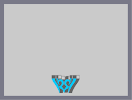Hover over the thumbnail for a full-size version.

Author RavenMac author:ravenmac dragon incomplete n-art nonplayable rated 2008-07-29 2008-07-29 4 by 9 people. \$Dragon Test#RavenMac#none#00000000000000000000000000000000000000000000000000000000000000000000000000000000000000000000000000000000000000000000000000000000000000000000000000000000000000000000000000000000000000000000000000000000000000000000000000000000000000000000000000000000000000000000000000000000000000000000000000000000000000000000000000000000000000000000000000000000000000000000000000000000000000000000000000000000000000000000000000000000000000000000000000000000000000000000000000000000000000000000000000000000000000000000000000000000000000000000000000000000000000000000000000000000000000000000000000000000000000000000000000000000000000000000000000000000000000000000000000000000000000000000000000000000000000000000000000000000000000000|8^364,548,1!1^365,547!8^365,548,1!1^366,547!1^367,548!8^420,548,1!1^421,547!8^421,548,1!1^422,547!1^423,548!8^364,547,1!1^365,546!8^365,547,1!1^366,546!1^367,547!8^420,547,1!1^421,546!8^421,547,1!1^422,546!1^423,547!8^364,546,1!1^365,545!8^365,546,1!1^366,545!8^366,546,1!1^367,545!1^368,546!8^392,546,1!1^393,545!8^393,546,1!1^394,545!1^395,546!8^419,546,1!1^420,545!8^420,546,1!1^421,545!8^421,546,1!1^422,545!1^423,546!8^364,545,1!1^365,544!8^365,545,1!1^366,544!8^366,545,1!1^367,544!1^368,545!8^391,545,1!1^392,544!8^392,545,1!1^393,544!8^393,545,1!1^394,544!8^394,545,1!1^395,544!1^396,545!8^419,545,1!1^420,544!8^420,545,1!1^421,544!8^421,545,1!1^422,544!1^423,545!8^364,544,1!1^365,543!8^365,544,1!1^366,543!8^366,544,1!1^367,543!1^368,544!8^390,544,1!1^391,543!8^391,544,1!1^392,543!8^392,544,1!1^393,543!8^393,544,1!1^394,543!8^394,544,1!1^395,543!8^395,544,1!1^396,543!1^397,544!8^419,544,1!1^420,543!8^420,544,1!1^421,543!8^421,544,1!1^422,543!1^423,544!8^364,543,1!1^365,542!8^365,543,1!1^366,542!8^366,543,1!1^367,542!1^368,543!8^390,543,1!1^391,542!8^391,543,1!1^392,542!8^392,543,1!1^393,542!8^393,543,1!1^394,542!8^394,543,1!1^395,542!8^395,543,1!1^396,542!1^397,543!8^419,543,1!1^420,542!8^420,543,1!1^421,542!8^421,543,1!1^422,542!1^423,543!8^363,542,1!1^364,541!8^364,542,1!1^365,541!8^365,542,1!1^366,541!8^366,542,1!1^367,541!1^368,542!8^389,542,1!1^390,541!8^390,542,1!1^391,541!8^391,542,1!1^392,541!8^392,542,1!1^393,541!8^393,542,1!1^394,541!8^394,542,1!1^395,541!8^395,542,1!1^396,541!8^396,542,1!1^397,541!1^398,542!8^419,542,1!1^420,541!8^420,542,1!1^421,541!8^421,542,1!1^422,541!8^422,542,1!1^423,541!1^424,542!8^363,541,1!1^364,540!8^364,541,1!1^365,540!8^365,541,1!1^366,540!8^366,541,1!1^367,540!1^368,541!8^389,541,1!1^390,540!8^390,541,1!1^391,540!8^391,541,1!1^392,540!8^392,541,1!1^393,540!8^393,541,1!1^394,540!8^394,541,1!1^395,540!8^395,541,1!1^396,540!8^396,541,1!1^397,540!1^398,541!8^419,541,1!1^420,540!8^420,541,1!1^421,540!8^421,541,1!1^422,540!8^422,541,1!1^423,540!1^424,541!8^362,540,1!1^363,539!8^363,540,1!1^364,539!8^364,540,1!1^365,539!8^365,540,1!1^366,539!8^366,540,1!1^367,539!1^368,540!8^389,540,1!1^390,539!8^390,540,1!1^391,539!8^391,540,1!1^392,539!8^392,540,1!1^393,539!8^393,540,1!1^394,539!8^394,540,1!1^395,539!8^395,540,1!1^396,539!8^396,540,1!1^397,539!1^398,540!8^419,540,1!1^420,539!8^420,540,1!1^421,539!8^421,540,1!1^422,539!8^422,540,1!1^423,539!8^423,540,1!1^424,539!1^425,540!8^362,539,1!1^363,538!8^363,539,1!1^364,538!8^364,539,1!1^365,538!8^365,539,1!1^366,538!8^366,539,1!1^367,538!1^368,539!8^389,539,1!1^390,538!8^390,539,1!1^391,538!8^391,539,1!1^392,538!8^392,539,1!1^393,538!8^393,539,1!1^394,538!8^394,539,1!1^395,538!8^395,539,1!1^396,538!8^396,539,1!1^397,538!1^398,539!8^419,539,1!1^420,538!8^420,539,1!1^421,538!8^421,539,1!1^422,538!8^422,539,1!1^423,538!8^423,539,1!1^424,538!1^425,539!8^362,538,1!1^363,537!8^363,538,1!1^364,537!8^364,538,1!1^365,537!8^365,538,1!1^366,537!8^366,538,1!1^367,537!1^368,538!8^388,538,1!1^389,537!8^389,538,1!1^390,537!8^390,538,1!1^391,537!8^391,538,1!1^392,537!8^392,538,1!1^393,537!8^393,538,1!1^394,537!8^394,538,1!1^395,537!8^395,538,1!1^396,537!8^396,538,1!1^397,537!8^397,538,1!1^398,537!1^399,538!8^419,538,1!1^420,537!8^420,538,1!1^421,537!8^421,538,1!1^422,537!8^422,538,1!1^423,537!8^423,538,1!1^424,537!1^425,538!8^361,537,1!1^362,536!8^362,537,1!1^363,536!8^363,537,1!1^364,536!8^364,537,1!1^365,536!8^365,537,1!1^366,536!8^366,537,1!1^367,536!1^368,537!8^388,537,1!1^389,536!8^389,537,1!1^390,536!8^390,537,1!1^391,536!8^391,537,1!1^392,536!8^392,537,1!1^393,536!8^393,537,1!1^394,536!8^394,537,1!1^395,536!8^395,537,1!1^396,536!8^396,537,1!1^397,536!8^397,537,1!1^398,536!1^399,537!8^419,537,1!1^420,536!8^420,537,1!1^421,536!8^421,537,1!1^422,536!8^422,537,1!1^423,536!8^423,537,1!1^424,536!8^424,537,1!1^425,536!1^426,537!8^361,536,1!1^362,535!8^362,536,1!1^363,535!8^363,536,1!1^364,535!8^364,536,1!1^365,535!8^365,536,1!1^366,535!8^366,536,1!1^367,535!1^368,536!8^388,536,1!1^389,535!8^389,536,1!1^390,535!8^390,536,1!1^391,535!8^391,536,1!1^392,535!8^392,536,1!1^393,535!8^393,536,1!1^394,535!8^394,536,1!1^395,535!8^395,536,1!1^396,535!8^396,536,1!1^397,535!8^397,536,1!1^398,535!1^399,536!8^419,536,1!1^420,535!8^420,536,1!1^421,535!8^421,536,1!1^422,535!8^422,536,1!1^423,535!8^423,536,1!1^424,535!8^424,536,1!1^425,535!1^426,536!8^360,535,1!1^361,534!8^361,535,1!1^362,534!8^362,535,1!1^363,534!8^363,535,1!1^364,534!8^364,535,1!1^365,534!8^365,535,1!1^366,534!8^366,535,1!1^367,534!1^368,535!8^388,535,1!1^389,534!8^389,535,1!1^390,534!8^390,535,1!1^391,534!8^391,535,1!1^392,534!8^392,535,1!1^393,534!8^393,535,1!1^394,534!8^394,535,1!1^395,534!8^395,535,1!1^396,534!8^396,535,1!1^397,534!8^397,535,1!1^398,534!1^399,535!8^419,535,1!1^420,534!8^420,535,1!1^421,534!8^421,535,1!1^422,534!8^422,535,1!1^423,534!8^423,535,1!1^424,534!8^424,535,1!1^425,534!8^425,535,1!1^426,534!1^427,535!8^360,534,1!1^361,533!8^361,534,1!1^362,533!8^362,534,1!1^363,533!8^363,534,1!1^364,533!8^364,534,1!1^365,533!8^365,534,1!1^366,533!8^366,534,1!1^367,533!1^368,534!8^387,534,1!1^388,533!8^388,534,1!1^389,533!8^389,534,1!1^390,533!8^390,534,1!1^391,533!8^391,534,1!1^392,533!8^392,534,1!1^393,533!8^393,534,1!1^394,533!8^394,534,1!1^395,533!8^395,534,1!1^396,533!8^396,534,1!1^397,533!8^397,534,1!1^398,533!8^398,534,1!1^399,533!1^400,534!8^419,534,1!1^420,533!8^420,534,1!1^421,533!8^421,534,1!1^422,533!8^422,534,1!1^423,533!8^423,534,1!1^424,533!8^424,534,1!1^425,533!8^425,534,1!1^426,533!1^427,534!8^360,533,1!1^361,532!8^361,533,1!1^362,532!8^362,533,1!1^363,532!8^363,533,1!1^364,532!8^364,533,1!1^365,532!8^365,533,1!1^366,532!8^366,533,1!1^367,532!1^368,533!8^387,533,1!1^388,532!8^388,533,1!1^389,532!8^389,533,1!1^390,532!8^390,533,1!1^391,532!8^391,533,1!1^392,532!8^392,533,1!1^393,532!8^393,533,1!1^394,532!8^394,533,1!1^395,532!8^395,533,1!1^396,532!8^396,533,1!1^397,532!8^397,533,1!1^398,532!8^398,533,1!1^399,532!1^400,533!8^419,533,1!1^420,532!8^420,533,1!1^421,532!8^421,533,1!1^422,532!8^422,533,1!1^423,532!8^423,533,1!1^424,532!8^424,533,1!1^425,532!8^425,533,1!1^426,532!1^427,533!8^359,532,1!1^360,531!8^360,532,1!1^361,531!8^361,532,1!1^362,531!8^362,532,1!1^363,531!8^363,532,1!1^364,531!8^364,532,1!1^365,531!8^365,532,1!1^366,531!8^366,532,1!1^367,531!1^368,532!8^386,532,1!1^387,531!8^387,532,1!1^388,531!8^388,532,1!1^389,531!8^389,532,1!1^390,531!8^390,532,1!1^391,531!8^391,532,1!1^392,531!8^392,532,1!1^393,531!8^393,532,1!1^394,531!8^394,532,1!1^395,531!8^395,532,1!1^396,531!8^396,532,1!1^397,531!8^397,532,1!1^398,531!8^398,532,1!1^399,531!8^399,532,1!1^400,531!1^401,532!8^419,532,1!1^420,531!8^420,532,1!1^421,531!8^421,532,1!1^422,531!8^422,532,1!1^423,531!8^423,532,1!1^424,531!8^424,532,1!1^425,531!8^425,532,1!1^426,531!8^426,532,1!1^427,531!1^428,532!8^359,531,1!1^360,530!8^360,531,1!1^361,530!8^361,531,1!1^362,530!8^362,531,1!1^363,530!8^363,531,1!1^364,530!8^364,531,1!1^365,530!8^365,531,1!1^366,530!8^366,531,1!1^367,530!1^368,531!8^386,531,1!1^387,530!8^387,531,1!1^388,530!8^388,531,1!1^389,530!8^389,531,1!1^390,530!8^390,531,1!1^391,530!8^391,531,1!1^392,530!8^392,531,1!1^393,530!8^393,531,1!1^394,530!8^394,531,1!1^395,530!8^395,531,1!1^396,530!8^396,531,1!1^397,530!8^397,531,1!1^398,530!8^398,531,1!1^399,530!8^399,531,1!1^400,530!1^401,531!8^419,531,1!1^420,530!8^420,531,1!1^421,530!8^421,531,1!1^422,530!8^422,531,1!1^423,530!8^423,531,1!1^424,530!8^424,531,1!1^425,530!8^425,531,1!1^426,530!8^426,531,1!1^427,530!1^428,531!8^358,530,1!1^359,529!8^359,530,1!1^360,529!8^360,530,1!1^361,529!8^361,530,1!1^362,529!8^362,530,1!1^363,529!8^363,530,1!1^364,529!8^364,530,1!1^365,529!8^365,530,1!1^366,529!1^367,530!8^385,530,1!1^386,529!8^386,530,1!1^387,529!8^387,530,1!1^388,529!8^388,530,1!1^389,529!8^389,530,1!1^390,529!8^390,530,1!1^391,529!8^391,530,1!1^392,529!8^392,530,1!1^393,529!8^393,530,1!1^394,529!8^394,530,1!1^395,529!8^395,530,1!1^396,529!8^396,530,1!1^397,529!8^397,530,1!1^398,529!8^398,530,1!1^399,529!8^399,530,1!1^400,529!8^400,530,1!1^401,529!1^402,530!8^420,530,1!1^421,529!8^421,530,1!1^422,529!8^422,530,1!1^423,529!8^423,530,1!1^424,529!8^424,530,1!1^425,529!8^425,530,1!1^426,529!8^426,530,1!1^427,529!8^427,530,1!1^428,529!1^429,530!8^358,529,1!1^359,528!8^359,529,1!1^360,528!8^360,529,1!1^361,528!8^361,529,1!1^362,528!8^362,529,1!1^363,528!8^363,529,1!1^364,528!8^364,529,1!1^365,528!8^365,529,1!1^366,528!1^367,529!8^384,529,1!1^385,528!8^385,529,1!1^386,528!8^386,529,1!1^387,528!8^387,529,1!1^388,528!8^388,529,1!1^389,528!8^389,529,1!1^390,528!8^390,529,1!1^391,528!8^391,529,1!1^392,528!8^392,529,1!1^393,528!8^393,529,1!1^394,528!8^394,529,1!1^395,528!8^395,529,1!1^396,528!8^396,529,1!1^397,528!8^397,529,1!1^398,528!8^398,529,1!1^399,528!8^399,529,1!1^400,528!8^400,529,1!1^401,528!8^401,529,1!1^402,528!1^403,529!8^420,529,1!1^421,528!8^421,529,1!1^422,528!8^422,529,1!1^423,528!8^423,529,1!1^424,528!8^424,529,1!1^425,528!8^425,529,1!1^426,528!8^426,529,1!1^427,528!8^427,529,1!1^428,528!1^429,529!8^357,528,1!1^358,527!8^358,528,1!1^359,527!8^359,528,1!1^360,527!8^360,528,1!1^361,527!8^361,528,1!1^362,527!8^362,528,1!1^363,527!8^363,528,1!1^364,527!8^364,528,1!1^365,527!8^365,528,1!1^366,527!1^367,528!8^383,528,1!1^384,527!8^384,528,1!1^385,527!8^385,528,1!1^386,527!8^386,528,1!1^387,527!8^387,528,1!1^388,527!8^388,528,1!1^389,527!8^389,528,1!1^390,527!8^390,528,1!1^391,527!8^391,528,1!1^392,527!8^392,528,1!1^393,527!8^393,528,1!1^394,527!8^394,528,1!1^395,527!8^395,528,1!1^396,527!8^396,528,1!1^397,527!8^397,528,1!1^398,527!8^398,528,1!1^399,527!8^399,528,1!1^400,527!8^400,528,1!1^401,527!8^401,528,1!1^402,527!8^402,528,1!1^403,527!1^404,528!8^420,528,1!1^421,527!8^421,528,1!1^422,527!8^422,528,1!1^423,527!8^423,528,1!1^424,527!8^424,528,1!1^425,527!8^425,528,1!1^426,527!8^426,528,1!1^427,527!8^427,528,1!1^428,527!8^428,528,1!1^429,527!1^430,528!8^357,527,1!1^358,526!8^358,527,1!1^359,526!8^359,527,1!1^360,526!8^360,527,1!1^361,526!8^361,527,1!1^362,526!8^362,527,1!1^363,526!8^363,527,1!1^364,526!8^364,527,1!1^365,526!8^365,527,1!1^366,526!1^367,527!8^381,527,1!1^382,526!8^382,527,1!1^383,526!8^383,527,1!1^384,526!8^384,527,1!1^385,526!8^385,527,1!1^386,526!8^386,527,1!1^387,526!8^387,527,1!1^388,526!8^388,527,1!1^389,526!8^389,527,1!1^390,526!8^390,527,1!1^391,526!8^391,527,1!1^392,526!1^393,527!8^394,527,1!1^395,526!8^395,527,1!1^396,526!8^396,527,1!1^397,526!8^397,527,1!1^398,526!8^398,527,1!1^399,526!8^399,527,1!1^400,526!8^400,527,1!1^401,526!8^401,527,1!1^402,526!8^402,527,1!1^403,526!8^403,527,1!1^404,526!8^404,527,1!1^405,526!1^406,527!8^420,527,1!1^421,526!8^421,527,1!1^422,526!8^422,527,1!1^423,526!8^423,527,1!1^424,526!8^424,527,1!1^425,526!8^425,527,1!1^426,526!8^426,527,1!1^427,526!8^427,527,1!1^428,526!8^428,527,1!1^429,526!1^430,527!8^356,526,1!1^357,525!8^357,526,1!1^358,525!8^358,526,1!1^359,525!8^359,526,1!1^360,525!8^360,526,1!1^361,525!8^361,526,1!1^362,525!8^362,526,1!1^363,525!8^363,526,1!1^364,525!8^364,526,1!1^365,525!8^365,526,1!1^366,525!1^367,526!8^379,526,1!1^380,525!8^380,526,1!1^381,525!8^381,526,1!1^382,525!8^382,526,1!1^383,525!8^383,526,1!1^384,525!8^384,526,1!1^385,525!8^385,526,1!1^386,525!8^386,526,1!1^387,525!8^387,526,1!1^388,525!8^388,526,1!1^389,525!8^389,526,1!1^390,525!8^390,526,1!1^391,525!1^392,526!8^395,526,1!1^396,525!8^396,526,1!1^397,525!8^397,526,1!1^398,525!8^398,526,1!1^399,525!8^399,526,1!1^400,525!8^400,526,1!1^401,525!8^401,526,1!1^402,525!8^402,526,1!1^403,525!8^403,526,1!1^404,525!8^404,526,1!1^405,525!8^405,526,1!1^406,525!8^406,526,1!1^407,525!1^408,526!8^420,526,1!1^421,525!8^421,526,1!1^422,525!8^422,526,1!1^423,525!8^423,526,1!1^424,525!8^424,526,1!1^425,525!8^425,526,1!1^426,525!8^426,526,1!1^427,525!8^427,526,1!1^428,525!8^428,526,1!1^429,525!8^429,526,1!1^430,525!1^431,526!8^356,525,1!1^357,524!8^357,525,1!1^358,524!8^358,525,1!1^359,524!8^359,525,1!1^360,524!8^360,525,1!1^361,524!8^361,525,1!1^362,524!8^362,525,1!1^363,524!8^363,525,1!1^364,524!8^364,525,1!1^365,524!8^365,525,1!1^366,524!1^367,525!8^378,525,1!1^379,524!8^379,525,1!1^380,524!8^380,525,1!1^381,524!8^381,525,1!1^382,524!8^382,525,1!1^383,524!8^383,525,1!1^384,524!8^384,525,1!1^385,524!8^385,525,1!1^386,524!8^386,525,1!1^387,524!8^387,525,1!1^388,524!8^388,525,1!1^389,524!8^389,525,1!1^390,524!8^390,525,1!1^391,524!1^392,525!8^395,525,1!1^396,524!8^396,525,1!1^397,524!8^397,525,1!1^398,524!8^398,525,1!1^399,524!8^399,525,1!1^400,524!8^400,525,1!1^401,524!8^401,525,1!1^402,524!8^402,525,1!1^403,524!8^403,525,1!1^404,524!8^404,525,1!1^405,524!8^405,525,1!1^406,524!8^406,525,1!1^407,524!8^407,525,1!1^408,524!1^409,525!8^420,525,1!1^421,524!8^421,525,1!1^422,524!8^422,525,1!1^423,524!8^423,525,1!1^424,524!8^424,525,1!1^425,524!8^425,525,1!1^426,524!8^426,525,1!1^427,524!8^427,525,1!1^428,524!8^428,525,1!1^429,524!8^429,525,1!1^430,524!1^431,525!8^355,524,1!1^356,523!8^356,524,1!1^357,523!8^357,524,1!1^358,523!8^358,524,1!1^359,523!8^359,524,1!1^360,523!8^360,524,1!1^361,523!8^361,524,1!1^362,523!8^362,524,1!1^363,523!8^363,524,1!1^364,523!8^364,524,1!1^365,523!1^366,524!8^376,524,1!1^377,523!8^377,524,1!1^378,523!8^378,524,1!1^379,523!8^379,524,1!1^380,523!8^380,524,1!1^381,523!8^381,524,1!1^382,523!8^382,524,1!1^383,523!8^383,524,1!1^384,523!8^384,524,1!1^385,523!8^385,524,1!1^386,523!8^386,524,1!1^387,523!8^387,524,1!1^388,523!8^388,524,1!1^389,523!8^389,524,1!1^390,523!1^391,524!8^396,524,1!1^397,523!8^397,524,1!1^398,523!8^398,524,1!1^399,523!8^399,524,1!1^400,523!8^400,524,1!1^401,523!8^401,524,1!1^402,523!8^402,524,1!1^403,523!8^403,524,1!1^404,523!8^404,524,1!1^405,523!8^405,524,1!1^406,523!8^406,524,1!1^407,523!8^407,524,1!1^408,523!8^408,524,1!1^409,523!8^409,524,1!1^410,523!1^411,524!8^421,524,1!1^422,523!8^422,524,1!1^423,523!8^423,524,1!1^424,523!8^424,524,1!1^425,523!8^425,524,1!1^426,523!8^426,524,1!1^427,523!8^427,524,1!1^428,523!8^428,524,1!1^429,523!8^429,524,1!1^430,523!8^430,524,1!1^431,523!1^432,524!8^354,523,1!1^355,522!8^355,523,1!1^356,522!8^356,523,1!1^357,522!8^357,523,1!1^358,522!8^358,523,1!1^359,522!8^359,523,1!1^360,522!8^360,523,1!1^361,522!8^361,523,1!1^362,522!8^362,523,1!1^363,522!8^363,523,1!1^364,522!8^364,523,1!1^365,522!1^366,523!8^374,523,1!1^375,522!8^375,523,1!1^376,522!8^376,523,1!1^377,522!8^377,523,1!1^378,522!8^378,523,1!1^379,522!8^379,523,1!1^380,522!8^380,523,1!1^381,522!8^381,523,1!1^382,522!8^382,523,1!1^383,522!8^383,523,1!1^384,522!8^384,523,1!1^385,522!8^385,523,1!1^386,522!8^386,523,1!1^387,522!8^387,523,1!1^388,522!8^388,523,1!1^389,522!1^390,523!8^397,523,1!1^398,522!8^398,523,1!1^399,522!8^399,523,1!1^400,522!8^400,523,1!1^401,522!8^401,523,1!1^402,522!8^402,523,1!1^403,522!8^403,523,1!1^404,522!8^404,523,1!1^405,522!8^405,523,1!1^406,522!8^406,523,1!1^407,522!8^407,523,1!1^408,522!8^408,523,1!1^409,522!8^409,523,1!1^410,522!8^410,523,1!1^411,522!8^411,523,1!1^412,522!1^413,523!8^421,523,1!1^422,522!8^422,523,1!1^423,522!8^423,523,1!1^424,522!8^424,523,1!1^425,522!8^425,523,1!1^426,522!8^426,523,1!1^427,522!8^427,523,1!1^428,522!8^428,523,1!1^429,522!8^429,523,1!1^430,522!8^430,523,1!1^431,522!8^431,523,1!1^432,522!1^433,523!8^354,522,1!1^355,521!8^355,522,1!1^356,521!8^356,522,1!1^357,521!8^357,522,1!1^358,521!8^358,522,1!1^359,521!8^359,522,1!1^360,521!8^360,522,1!1^361,521!8^361,522,1!1^362,521!8^362,522,1!1^363,521!8^363,522,1!1^364,521!8^364,522,1!1^365,521!1^366,522!8^372,522,1!1^373,521!8^373,522,1!1^374,521!8^374,522,1!1^375,521!8^375,522,1!1^376,521!8^376,522,1!1^377,521!8^377,522,1!1^378,521!8^378,522,1!1^379,521!8^379,522,1!1^380,521!8^380,522,1!1^381,521!8^381,522,1!1^382,521!8^382,522,1!1^383,521!8^383,522,1!1^384,521!8^384,522,1!1^385,521!8^385,522,1!1^386,521!8^386,522,1!1^387,521!8^387,522,1!1^388,521!1^389,522!8^398,522,1!1^399,521!8^399,522,1!1^400,521!8^400,522,1!1^401,521!8^401,522,1!1^402,521!8^402,522,1!1^403,521!8^403,522,1!1^404,521!8^404,522,1!1^405,521!8^405,522,1!1^406,521!8^406,522,1!1^407,521!8^407,522,1!1^408,521!8^408,522,1!1^409,521!8^409,522,1!1^410,521!8^410,522,1!1^411,521!8^411,522,1!1^412,521!8^412,522,1!1^413,521!8^413,522,1!1^414,521!1^415,522!8^421,522,1!1^422,521!8^422,522,1!1^423,521!8^423,522,1!1^424,521!8^424,522,1!1^425,521!8^425,522,1!1^426,521!8^426,522,1!1^427,521!8^427,522,1!1^428,521!8^428,522,1!1^429,521!8^429,522,1!1^430,521!8^430,522,1!1^431,521!8^431,522,1!1^432,521!1^433,522!8^353,521,1!1^354,520!8^354,521,1!1^355,520!8^355,521,1!1^356,520!8^356,521,1!1^357,520!8^357,521,1!1^358,520!8^358,521,1!1^359,520!8^359,521,1!1^360,520!8^360,521,1!1^361,520!8^361,521,1!1^362,520!8^362,521,1!1^363,520!8^363,521,1!1^364,520!8^364,521,1!1^365,520!1^366,521!8^371,521,1!1^372,520!8^372,521,1!1^373,520!8^373,521,1!1^374,520!8^374,521,1!1^375,520!8^375,521,1!1^376,520!8^376,521,1!1^377,520!8^377,521,1!1^378,520!8^378,521,1!1^379,520!8^379,521,1!1^380,520!8^380,521,1!1^381,520!8^381,521,1!1^382,520!8^382,521,1!1^383,520!8^383,521,1!1^384,520!8^384,521,1!1^385,520!8^385,521,1!1^386,520!8^386,521,1!1^387,520!8^387,521,1!1^388,520!1^389,521!8^398,521,1!1^399,520!8^399,521,1!1^400,520!8^400,521,1!1^401,520!8^401,521,1!1^402,520!8^402,521,1!1^403,520!8^403,521,1!1^404,520!8^404,521,1!1^405,520!8^405,521,1!1^406,520!8^406,521,1!1^407,520!8^407,521,1!1^408,520!8^408,521,1!1^409,520!8^409,521,1!1^410,520!8^410,521,1!1^411,520!8^411,521,1!1^412,520!8^412,521,1!1^413,520!8^413,521,1!1^414,520!8^414,521,1!1^415,520!1^416,521!8^421,521,1!1^422,520!8^422,521,1!1^423,520!8^423,521,1!1^424,520!8^424,521,1!1^425,520!8^425,521,1!1^426,520!8^426,521,1!1^427,520!8^427,521,1!1^428,520!8^428,521,1!1^429,520!8^429,521,1!1^430,520!8^430,521,1!1^431,520!8^431,521,1!1^432,520!8^432,521,1!1^433,520!1^434,521!8^353,520,1!1^354,519!8^354,520,1!1^355,519!8^355,520,1!1^356,519!8^356,520,1!1^357,519!8^357,520,1!1^358,519!8^358,520,1!1^359,519!8^359,520,1!1^360,519!8^360,520,1!1^361,519!8^361,520,1!1^362,519!8^362,520,1!1^363,519!8^363,520,1!1^364,519!1^365,520!8^370,520,1!1^371,519!8^371,520,1!1^372,519!8^372,520,1!1^373,519!8^373,520,1!1^374,519!8^374,520,1!1^375,519!8^375,520,1!1^376,519!8^376,520,1!1^377,519!8^377,520,1!1^378,519!8^378,520,1!1^379,519!8^379,520,1!1^380,519!8^380,520,1!1^381,519!8^381,520,1!1^382,519!8^382,520,1!1^383,519!8^383,520,1!1^384,519!8^384,520,1!1^385,519!8^385,520,1!1^386,519!8^386,520,1!1^387,519!1^388,520!8^399,520,1!1^400,519!8^400,520,1!1^401,519!8^401,520,1!1^402,519!8^402,520,1!1^403,519!8^403,520,1!1^404,519!8^404,520,1!1^405,519!8^405,520,1!1^406,519!8^406,520,1!1^407,519!8^407,520,1!1^408,519!8^408,520,1!1^409,519!8^409,520,1!1^410,519!8^410,520,1!1^411,519!8^411,520,1!1^412,519!8^412,520,1!1^413,519!8^413,520,1!1^414,519!8^414,520,1!1^415,519!8^415,520,1!1^416,519!1^417,520!8^422,520,1!1^423,519!8^423,520,1!1^424,519!8^424,520,1!1^425,519!8^425,520,1!1^426,519!8^426,520,1!1^427,519!8^427,520,1!1^428,519!8^428,520,1!1^429,519!8^429,520,1!1^430,519!8^430,520,1!1^431,519!8^431,520,1!1^432,519!8^432,520,1!1^433,519!1^434,520!8^352,519,1!1^353,518!8^353,519,1!1^354,518!8^354,519,1!1^355,518!8^355,519,1!1^356,518!8^356,519,1!1^357,518!8^357,519,1!1^358,518!8^358,519,1!1^359,518!8^359,519,1!1^360,518!8^360,519,1!1^361,518!8^361,519,1!1^362,518!8^362,519,1!1^363,518!8^363,519,1!1^364,518!1^365,519!8^369,519,1!1^370,518!8^370,519,1!1^371,518!8^371,519,1!1^372,518!8^372,519,1!1^373,518!8^373,519,1!1^374,518!8^374,519,1!1^375,518!8^375,519,1!1^376,518!8^376,519,1!1^377,518!8^377,519,1!1^378,518!8^378,519,1!1^379,518!8^379,519,1!1^380,518!8^380,519,1!1^381,518!8^381,519,1!1^382,518!8^382,519,1!1^383,518!8^383,519,1!1^384,518!8^384,519,1!1^385,518!8^385,519,1!1^386,518!1^387,519!8^400,519,1!1^401,518!8^401,519,1!1^402,518!8^402,519,1!1^403,518!8^403,519,1!1^404,518!8^404,519,1!1^405,518!8^405,519,1!1^406,518!8^406,519,1!1^407,518!8^407,519,1!1^408,518!8^408,519,1!1^409,518!8^409,519,1!1^410,518!8^410,519,1!1^411,518!8^411,519,1!1^412,518!8^412,519,1!1^413,518!8^413,519,1!1^414,518!8^414,519,1!1^415,518!8^415,519,1!1^416,518!8^416,519,1!1^417,518!1^418,519!8^422,519,1!1^423,518!8^423,519,1!1^424,518!8^424,519,1!1^425,518!8^425,519,1!1^426,518!8^426,519,1!1^427,518!8^427,519,1!1^428,518!8^428,519,1!1^429,518!8^429,519,1!1^430,518!8^430,519,1!1^431,518!8^431,519,1!1^432,518!8^432,519,1!1^433,518!8^433,519,1!1^434,518!1^435,519!8^352,518,1!1^353,517!8^353,518,1!1^354,517!8^354,518,1!1^355,517!8^355,518,1!1^356,517!8^356,518,1!1^357,517!8^357,518,1!1^358,517!8^358,518,1!1^359,517!8^359,518,1!1^360,517!8^360,518,1!1^361,517!8^361,518,1!1^362,517!8^362,518,1!1^363,517!8^363,518,1!1^364,517!1^365,518!8^368,518,1!1^369,517!8^369,518,1!1^370,517!8^370,518,1!1^371,517!8^371,518,1!1^372,517!8^372,518,1!1^373,517!8^373,518,1!1^374,517!8^374,518,1!1^375,517!8^375,518,1!1^376,517!8^376,518,1!1^377,517!8^377,518,1!1^378,517!8^378,518,1!1^379,517!8^379,518,1!1^380,517!8^380,518,1!1^381,517!8^381,518,1!1^382,517!8^382,518,1!1^383,517!8^383,518,1!1^384,517!8^384,518,1!1^385,517!8^385,518,1!1^386,517!1^387,518!8^400,518,1!1^401,517!8^401,518,1!1^402,517!8^402,518,1!1^403,517!8^403,518,1!1^404,517!8^404,518,1!1^405,517!8^405,518,1!1^406,517!8^406,518,1!1^407,517!8^407,518,1!1^408,517!8^408,518,1!1^409,517!8^409,518,1!1^410,517!8^410,518,1!1^411,517!8^411,518,1!1^412,517!8^412,518,1!1^413,517!8^413,518,1!1^414,517!8^414,518,1!1^415,517!8^415,518,1!1^416,517!8^416,518,1!1^417,517!8^417,518,1!1^418,517!1^419,518!8^422,518,1!1^423,517!8^423,518,1!1^424,517!8^424,518,1!1^425,517!8^425,518,1!1^426,517!8^426,518,1!1^427,517!8^427,518,1!1^428,517!8^428,518,1!1^429,517!8^429,518,1!1^430,517!8^430,518,1!1^431,517!8^431,518,1!1^432,517!8^432,518,1!1^433,517!8^433,518,1!1^434,517!1^435,518!8^351,517,1!1^352,516!8^352,517,1!1^353,516!8^353,517,1!1^354,516!8^354,517,1!1^355,516!8^355,517,1!1^356,516!8^356,517,1!1^357,516!8^357,517,1!1^358,516!8^358,517,1!1^359,516!8^359,517,1!1^360,516!8^360,517,1!1^361,516!8^361,517,1!1^362,516!8^362,517,1!1^363,516!8^363,517,1!1^364,516!1^365,517!8^367,517,1!1^368,516!8^368,517,1!1^369,516!8^369,517,1!1^370,516!8^370,517,1!1^371,516!8^371,517,1!1^372,516!8^372,517,1!1^373,516!8^373,517,1!1^374,516!8^374,517,1!1^375,516!8^375,517,1!1^376,516!8^376,517,1!1^377,516!8^377,517,1!1^378,516!8^378,517,1!1^379,516!8^379,517,1!1^380,516!8^380,517,1!1^381,516!8^381,517,1!1^382,516!8^382,517,1!1^383,516!8^383,517,1!1^384,516!8^384,517,1!1^385,516!1^386,517!8^401,517,1!1^402,516!8^402,517,1!1^403,516!8^403,517,1!1^404,516!8^404,517,1!1^405,516!8^405,517,1!1^406,516!8^406,517,1!1^407,516!8^407,517,1!1^408,516!8^408,517,1!1^409,516!8^409,517,1!1^410,516!8^410,517,1!1^411,516!8^411,517,1!1^412,516!8^412,517,1!1^413,516!8^413,517,1!1^414,516!8^414,517,1!1^415,516!8^415,517,1!1^416,516!8^416,517,1!1^417,516!8^417,517,1!1^418,516!8^418,517,1!1^419,516!1^420,517!8^422,517,1!1^423,516!8^423,517,1!1^424,516!8^424,517,1!1^425,516!8^425,517,1!1^426,516!8^426,517,1!1^427,516!8^427,517,1!1^428,516!8^428,517,1!1^429,516!8^429,517,1!1^430,516!8^430,517,1!1^431,516!8^431,517,1!1^432,516!8^432,517,1!1^433,516!8^433,517,1!1^434,516!8^434,517,1!1^435,516!1^436,517!8^351,516,1!1^352,515!8^352,516,1!1^353,515!8^353,516,1!1^354,515!8^354,516,1!1^355,515!8^355,516,1!1^356,515!8^356,516,1!1^357,515!8^357,516,1!1^358,515!8^358,516,1!1^359,515!8^359,516,1!1^360,515!8^360,516,1!1^361,515!8^361,516,1!1^362,515!8^362,516,1!1^363,515!1^364,516!8^367,516,1!1^368,515!8^368,516,1!1^369,515!8^369,516,1!1^370,515!8^370,516,1!1^371,515!8^371,516,1!1^372,515!8^372,516,1!1^373,515!8^373,516,1!1^374,515!8^374,516,1!1^375,515!8^375,516,1!1^376,515!8^376,516,1!1^377,515!8^377,516,1!1^378,515!8^378,516,1!1^379,515!8^379,516,1!1^380,515!8^380,516,1!1^381,515!8^381,516,1!1^382,515!8^382,516,1!1^383,515!8^383,516,1!1^384,515!1^385,516!8^402,516,1!1^403,515!8^403,516,1!1^404,515!8^404,516,1!1^405,515!8^405,516,1!1^406,515!8^406,516,1!1^407,515!8^407,516,1!1^408,515!8^408,516,1!1^409,515!8^409,516,1!1^410,515!8^410,516,1!1^411,515!8^411,516,1!1^412,515!8^412,516,1!1^413,515!8^413,516,1!1^414,515!8^414,516,1!1^415,515!8^415,516,1!1^416,515!8^416,516,1!1^417,515!8^417,516,1!1^418,515!8^418,516,1!1^419,515!1^420,516!8^423,516,1!1^424,515!8^424,516,1!1^425,515!8^425,516,1!1^426,515!8^426,516,1!1^427,515!8^427,516,1!1^428,515!8^428,516,1!1^429,515!8^429,516,1!1^430,515!8^430,516,1!1^431,515!8^431,516,1!1^432,515!8^432,516,1!1^433,515!8^433,516,1!1^434,515!8^434,516,1!1^435,515!1^436,516!8^350,515,1!1^351,514!8^351,515,1!1^352,514!8^352,515,1!1^353,514!8^353,515,1!1^354,514!8^354,515,1!1^355,514!8^355,515,1!1^356,514!8^356,515,1!1^357,514!8^357,515,1!1^358,514!8^358,515,1!1^359,514!8^359,515,1!1^360,514!8^360,515,1!1^361,514!8^361,515,1!1^362,514!8^362,515,1!1^363,514!1^364,515!8^366,515,1!1^367,514!8^367,515,1!1^368,514!8^368,515,1!1^369,514!8^369,515,1!1^370,514!8^370,515,1!1^371,514!8^371,515,1!1^372,514!8^372,515,1!1^373,514!8^373,515,1!1^374,514!8^374,515,1!1^375,514!8^375,515,1!1^376,514!8^376,515,1!1^377,514!8^377,515,1!1^378,514!8^378,515,1!1^379,514!8^379,515,1!1^380,514!8^380,515,1!1^381,514!8^381,515,1!1^382,514!8^382,515,1!1^383,514!1^384,515!8^403,515,1!1^404,514!8^404,515,1!1^405,514!8^405,515,1!1^406,514!8^406,515,1!1^407,514!8^407,515,1!1^408,514!8^408,515,1!1^409,514!8^409,515,1!1^410,514!8^410,515,1!1^411,514!8^411,515,1!1^412,514!8^412,515,1!1^413,514!8^413,515,1!1^414,514!8^414,515,1!1^415,514!8^415,515,1!1^416,514!8^416,515,1!1^417,514!8^417,515,1!1^418,514!8^418,515,1!1^419,514!8^419,515,1!1^420,514!1^421,515!8^423,515,1!1^424,514!8^424,515,1!1^425,514!8^425,515,1!1^426,514!8^426,515,1!1^427,514!8^427,515,1!1^428,514!8^428,515,1!1^429,514!8^429,515,1!1^430,514!8^430,515,1!1^431,514!8^431,515,1!1^432,514!8^432,515,1!1^433,514!8^433,515,1!1^434,514!8^434,515,1!1^435,514!8^435,515,1!1^436,514!1^437,515!8^350,514,1!1^351,513!8^351,514,1!1^352,513!8^352,514,1!1^353,513!8^353,514,1!1^354,513!8^354,514,1!1^355,513!8^355,514,1!1^356,513!8^356,514,1!1^357,513!8^357,514,1!1^358,513!8^358,514,1!1^359,513!8^359,514,1!1^360,513!8^360,514,1!1^361,513!8^361,514,1!1^362,513!8^362,514,1!1^363,513!1^364,514!8^365,514,1!1^366,513!8^366,514,1!1^367,513!8^367,514,1!1^368,513!8^368,514,1!1^369,513!8^369,514,1!1^370,513!8^370,514,1!1^371,513!8^371,514,1!1^372,513!8^372,514,1!1^373,513!8^373,514,1!1^374,513!8^374,514,1!1^375,513!8^375,514,1!1^376,513!8^376,514,1!1^377,513!8^377,514,1!1^378,513!8^378,514,1!1^379,513!8^379,514,1!1^380,513!8^380,514,1!1^381,513!8^381,514,1!1^382,513!1^383,514!8^404,514,1!1^405,513!8^405,514,1!1^406,513!8^406,514,1!1^407,513!8^407,514,1!1^408,513!8^408,514,1!1^409,513!8^409,514,1!1^410,513!8^410,514,1!1^411,513!8^411,514,1!1^412,513!8^412,514,1!1^413,513!8^413,514,1!1^414,513!8^414,514,1!1^415,513!8^415,514,1!1^416,513!8^416,514,1!1^417,513!8^417,514,1!1^418,513!8^418,514,1!1^419,513!8^419,514,1!1^420,513!8^420,514,1!1^421,513!1^422,514!8^423,514,1!1^424,513!8^424,514,1!1^425,513!8^425,514,1!1^426,513!8^426,514,1!1^427,513!8^427,514,1!1^428,513!8^428,514,1!1^429,513!8^429,514,1!1^430,513!8^430,514,1!1^431,513!8^431,514,1!1^432,513!8^432,514,1!1^433,513!8^433,514,1!1^434,513!8^434,514,1!1^435,513!8^435,514,1!1^436,513!1^437,514!8^349,513,1!1^350,512!8^350,513,1!1^351,512!8^351,513,1!1^352,512!8^352,513,1!1^353,512!8^353,513,1!1^354,512!8^354,513,1!1^355,512!8^355,513,1!1^356,512!8^356,513,1!1^357,512!8^357,513,1!1^358,512!8^358,513,1!1^359,512!8^359,513,1!1^360,512!8^360,513,1!1^361,512!8^361,513,1!1^362,512!1^363,513!8^365,513,1!1^366,512!8^366,513,1!1^367,512!8^367,513,1!1^368,512!8^368,513,1!1^369,512!8^369,513,1!1^370,512!8^370,513,1!1^371,512!8^371,513,1!1^372,512!8^372,513,1!1^373,512!8^373,513,1!1^374,512!8^374,513,1!1^375,512!8^375,513,1!1^376,512!8^376,513,1!1^377,512!8^377,513,1!1^378,512!8^378,513,1!1^379,512!8^379,513,1!1^380,512!8^380,513,1!1^381,512!8^381,513,1!1^382,512!1^383,513!8^404,513,1!1^405,512!8^405,513,1!1^406,512!8^406,513,1!1^407,512!8^407,513,1!1^408,512!8^408,513,1!1^409,512!8^409,513,1!1^410,512!8^410,513,1!1^411,512!8^411,513,1!1^412,512!8^412,513,1!1^413,512!8^413,513,1!1^414,512!8^414,513,1!1^415,512!8^415,513,1!1^416,512!8^416,513,1!1^417,512!8^417,513,1!1^418,512!8^418,513,1!1^419,512!8^419,513,1!1^420,512!8^420,513,1!1^421,512!1^422,513!8^424,513,1!1^425,512!8^425,513,1!1^426,512!8^426,513,1!1^427,512!8^427,513,1!1^428,512!8^428,513,1!1^429,512!8^429,513,1!1^430,512!8^430,513,1!1^431,512!8^431,513,1!1^432,512!8^432,513,1!1^433,512!8^433,513,1!1^434,512!8^434,513,1!1^435,512!8^435,513,1!1^436,512!8^436,513,1!1^437,512!1^438,513!8^348,512,1!1^349,511!8^349,512,1!1^350,511!8^350,512,1!1^351,511!8^351,512,1!1^352,511!8^352,512,1!1^353,511!8^353,512,1!1^354,511!8^354,512,1!1^355,511!8^355,512,1!1^356,511!8^356,512,1!1^357,511!8^357,512,1!1^358,511!8^358,512,1!1^359,511!8^359,512,1!1^360,511!8^360,512,1!1^361,511!8^361,512,1!1^362,511!1^363,512!8^364,512,1!1^365,511!8^365,512,1!1^366,511!8^366,512,1!1^367,511!8^367,512,1!1^368,511!8^368,512,1!1^369,511!8^369,512,1!1^370,511!8^370,512,1!1^371,511!8^371,512,1!1^372,511!8^372,512,1!1^373,511!8^373,512,1!1^374,511!8^374,512,1!1^375,511!8^375,512,1!1^376,511!8^376,512,1!1^377,511!8^377,512,1!1^378,511!8^378,512,1!1^379,511!8^379,512,1!1^380,511!8^380,512,1!1^381,511!8^381,512,1!1^382,511!1^383,512!8^404,512,1!1^405,511!8^405,512,1!1^406,511!8^406,512,1!1^407,511!8^407,512,1!1^408,511!8^408,512,1!1^409,511!8^409,512,1!1^410,511!8^410,512,1!1^411,511!8^411,512,1!1^412,511!8^412,512,1!1^413,511!8^413,512,1!1^414,511!8^414,512,1!1^415,511!8^415,512,1!1^416,511!8^416,512,1!1^417,511!8^417,512,1!1^418,511!8^418,512,1!1^419,511!8^419,512,1!1^420,511!8^420,512,1!1^421,511!8^421,512,1!1^422,511!1^423,512!8^424,512,1!1^425,511!8^425,512,1!1^426,511!8^426,512,1!1^427,511!8^427,512,1!1^428,511!8^428,512,1!1^429,511!8^429,512,1!1^430,511!8^430,512,1!1^431,511!8^431,512,1!1^432,511!8^432,512,1!1^433,511!8^433,512,1!1^434,511!8^434,512,1!1^435,511!8^435,512,1!1^436,511!8^436,512,1!1^437,511!8^437,512,1!1^438,511!1^439,512!8^348,511,1!1^349,510!8^349,511,1!1^350,510!8^350,511,1!1^351,510!8^351,511,1!1^352,510!8^352,511,1!1^353,510!8^353,511,1!1^354,510!8^354,511,1!1^355,510!8^355,511,1!1^356,510!8^356,511,1!1^357,510!8^357,511,1!1^358,510!8^358,511,1!1^359,510!8^359,511,1!1^360,510!8^360,511,1!1^361,510!1^362,511!8^364,511,1!1^365,510!8^365,511,1!1^366,510!8^366,511,1!1^367,510!8^367,511,1!1^368,510!8^368,511,1!1^369,510!8^369,511,1!1^370,510!8^370,511,1!1^371,510!8^371,511,1!1^372,510!8^372,511,1!1^373,510!8^373,511,1!1^374,510!8^374,511,1!1^375,510!8^375,511,1!1^376,510!8^376,511,1!1^377,510!8^377,511,1!1^378,510!8^378,511,1!1^379,510!8^379,511,1!1^380,510!8^380,511,1!1^381,510!1^382,511!8^405,511,1!1^406,510!8^406,511,1!1^407,510!8^407,511,1!1^408,510!8^408,511,1!1^409,510!8^409,511,1!1^410,510!8^410,511,1!1^411,510!8^411,511,1!1^412,510!8^412,511,1!1^413,510!8^413,511,1!1^414,510!8^414,511,1!1^415,510!8^415,511,1!1^416,510!8^416,511,1!1^417,510!8^417,511,1!1^418,510!8^418,511,1!1^419,510!8^419,511,1!1^420,510!8^420,511,1!1^421,510!8^421,511,1!1^422,510!1^423,511!8^425,511,1!1^426,510!8^426,511,1!1^427,510!8^427,511,1!1^428,510!8^428,511,1!1^429,510!8^429,511,1!1^430,510!8^430,511,1!1^431,510!8^431,511,1!1^432,510!8^432,511,1!1^433,510!8^433,511,1!1^434,510!8^434,511,1!1^435,510!8^435,511,1!1^436,510!8^436,511,1!1^437,510!8^437,511,1!1^438,510!1^439,511!8^347,510,1!1^348,509!8^348,510,1!1^349,509!8^349,510,1!1^350,509!8^350,510,1!1^351,509!8^351,510,1!1^352,509!8^352,510,1!1^353,509!8^353,510,1!1^354,509!8^354,510,1!1^355,509!8^355,510,1!1^356,509!8^356,510,1!1^357,509!8^357,510,1!1^358,509!8^358,510,1!1^359,509!8^359,510,1!1^360,509!8^360,510,1!1^361,509!1^362,510!8^363,510,1!1^364,509!8^364,510,1!1^365,509!8^365,510,1!1^366,509!8^366,510,1!1^367,509!8^367,510,1!1^368,509!8^368,510,1!1^369,509!8^369,510,1!1^370,509!8^370,510,1!1^371,509!8^371,510,1!1^372,509!8^372,510,1!1^373,509!8^373,510,1!1^374,509!8^374,510,1!1^375,509!8^375,510,1!1^376,509!8^376,510,1!1^377,509!8^377,510,1!1^378,509!8^378,510,1!1^379,509!8^379,510,1!1^380,509!8^380,510,1!1^381,509!1^382,510!8^405,510,1!1^406,509!8^406,510,1!1^407,509!8^407,510,1!1^408,509!8^408,510,1!1^409,509!8^409,510,1!1^410,509!8^410,510,1!1^411,509!8^411,510,1!1^412,509!8^412,510,1!1^413,509!8^413,510,1!1^414,509!8^414,510,1!1^415,509!8^415,510,1!1^416,509!8^416,510,1!1^417,509!8^417,510,1!1^418,509!8^418,510,1!1^419,509!8^419,510,1!1^420,509!8^420,510,1!1^421,509!8^421,510,1!1^422,509!8^422,510,1!1^423,509!1^424,510!8^425,510,1!1^426,509!8^426,510,1!1^427,509!8^427,510,1!1^428,509!8^428,510,1!1^429,509!8^429,510,1!1^430,509!8^430,510,1!1^431,509!8^431,510,1!1^432,509!8^432,510,1!1^433,509!8^433,510,1!1^434,509!8^434,510,1!1^435,509!8^435,510,1!1^436,509!8^436,510,1!1^437,509!8^437,510,1!1^438,509!8^438,510,1!1^439,509!1^440,510!8^346,509,1!1^347,508!8^347,509,1!1^348,508!8^348,509,1!1^349,508!8^349,509,1!1^350,508!8^350,509,1!1^351,508!8^351,509,1!1^352,508!8^352,509,1!1^353,508!8^353,509,1!1^354,508!8^354,509,1!1^355,508!8^355,509,1!1^356,508!8^356,509,1!1^357,508!8^357,509,1!1^358,508!8^358,509,1!1^359,508!8^359,509,1!1^360,508!1^361,509!8^363,509,1!1^364,508!8^364,509,1!1^365,508!8^365,509,1!1^366,508!8^366,509,1!1^367,508!8^367,509,1!1^368,508!8^368,509,1!1^369,508!8^369,509,1!1^370,508!8^370,509,1!1^371,508!8^371,509,1!1^372,508!8^372,509,1!1^373,508!8^373,509,1!1^374,508!8^374,509,1!1^375,508!8^375,509,1!1^376,508!8^376,509,1!1^377,508!8^377,509,1!1^378,508!8^378,509,1!1^379,508!8^379,509,1!1^380,508!1^381,509!8^406,509,1!1^407,508!8^407,509,1!1^408,508!8^408,509,1!1^409,508!8^409,509,1!1^410,508!8^410,509,1!1^411,508!8^411,509,1!1^412,508!8^412,509,1!1^413,508!8^413,509,1!1^414,508!8^414,509,1!1^415,508!8^415,509,1!1^416,508!8^416,509,1!1^417,508!8^417,509,1!1^418,508!8^418,509,1!1^419,508!8^419,509,1!1^420,508!8^420,509,1!1^421,508!8^421,509,1!1^422,508!8^422,509,1!1^423,508!1^424,509!8^426,509,1!1^427,508!8^427,509,1!1^428,508!8^428,509,1!1^429,508!8^429,509,1!1^430,508!8^430,509,1!1^431,508!8^431,509,1!1^432,508!8^432,509,1!1^433,508!8^433,509,1!1^434,508!8^434,509,1!1^435,508!8^435,509,1!1^436,508!8^436,509,1!1^437,508!8^437,509,1!1^438,508!8^438,509,1!1^439,508!8^439,509,1!1^440,508!1^441,509!8^346,508,1!1^347,507!8^347,508,1!1^348,507!8^348,508,1!1^349,507!8^349,508,1!1^350,507!8^350,508,1!1^351,507!8^351,508,1!1^352,507!8^352,508,1!1^353,507!8^353,508,1!1^354,507!8^354,508,1!1^355,507!8^355,508,1!1^356,507!8^356,508,1!1^357,507!8^357,508,1!1^358,507!8^358,508,1!1^359,507!8^359,508,1!1^360,507!1^361,508!8^362,508,1!1^363,507!8^363,508,1!1^364,507!8^364,508,1!1^365,507!8^365,508,1!1^366,507!8^366,508,1!1^367,507!8^367,508,1!1^368,507!8^368,508,1!1^369,507!8^369,508,1!1^370,507!8^370,508,1!1^371,507!8^371,508,1!1^372,507!8^372,508,1!1^373,507!8^373,508,1!1^374,507!8^374,508,1!1^375,507!8^375,508,1!1^376,507!8^376,508,1!1^377,507!8^377,508,1!1^378,507!8^378,508,1!1^379,507!8^379,508,1!1^380,507!8^380,508,1!1^381,507!1^382,508!8^405,508,1!1^406,507!8^406,508,1!1^407,507!8^407,508,1!1^408,507!8^408,508,1!1^409,507!8^409,508,1!1^410,507!8^410,508,1!1^411,507!8^411,508,1!1^412,507!8^412,508,1!1^413,507!8^413,508,1!1^414,507!8^414,508,1!1^415,507!8^415,508,1!1^416,507!8^416,508,1!1^417,507!8^417,508,1!1^418,507!8^418,508,1!1^419,507!8^419,508,1!1^420,507!8^420,508,1!1^421,507!8^421,508,1!1^422,507!8^422,508,1!1^423,507!8^423,508,1!1^424,507!1^425,508!8^426,508,1!1^427,507!8^427,508,1!1^428,507!8^428,508,1!1^429,507!8^429,508,1!1^430,507!8^430,508,1!1^431,507!8^431,508,1!1^432,507!8^432,508,1!1^433,507!8^433,508,1!1^434,507!8^434,508,1!1^435,507!8^435,508,1!1^436,507!8^436,508,1!1^437,507!8^437,508,1!1^438,507!8^438,508,1!1^439,507!8^439,508,1!1^440,507!1^441,508!8^345,507,1!1^346,506!8^346,507,1!1^347,506!8^347,507,1!1^348,506!8^348,507,1!1^349,506!8^349,507,1!1^350,506!8^350,507,1!1^351,506!8^351,507,1!1^352,506!8^352,507,1!1^353,506!8^353,507,1!1^354,506!8^354,507,1!1^355,506!8^355,507,1!1^356,506!8^356,507,1!1^357,506!8^357,507,1!1^358,506!8^358,507,1!1^359,506!1^360,507!8^362,507,1!1^363,506!8^363,507,1!1^364,506!8^364,507,1!1^365,506!8^365,507,1!1^366,506!8^366,507,1!1^367,506!8^367,507,1!1^368,506!8^368,507,1!1^369,506!8^369,507,1!1^370,506!8^370,507,1!1^371,506!8^371,507,1!1^372,506!8^372,507,1!1^373,506!8^373,507,1!1^374,506!8^374,507,1!1^375,506!8^375,507,1!1^376,506!8^376,507,1!1^377,506!8^377,507,1!1^378,506!8^378,507,1!1^379,506!8^379,507,1!1^380,506!8^380,507,1!1^381,506!8^381,507,1!1^382,506!1^383,507!8^404,507,1!1^405,506!8^405,507,1!1^406,506!8^406,507,1!1^407,506!8^407,507,1!1^408,506!8^408,507,1!1^409,506!8^409,507,1!1^410,506!8^410,507,1!1^411,506!8^411,507,1!1^412,506!8^412,507,1!1^413,506!8^413,507,1!1^414,506!8^414,507,1!1^415,506!8^415,507,1!1^416,506!8^416,507,1!1^417,506!8^417,507,1!1^418,506!8^418,507,1!1^419,506!8^419,507,1!1^420,506!8^420,507,1!1^421,506!8^421,507,1!1^422,506!8^422,507,1!1^423,506!8^423,507,1!1^424,506!1^425,507!8^427,507,1!1^428,506!8^428,507,1!1^429,506!8^429,507,1!1^430,506!8^430,507,1!1^431,506!8^431,507,1!1^432,506!8^432,507,1!1^433,506!8^433,507,1!1^434,506!8^434,507,1!1^435,506!8^435,507,1!1^436,506!8^436,507,1!1^437,506!8^437,507,1!1^438,506!8^438,507,1!1^439,506!8^439,507,1!1^440,506!8^440,507,1!1^441,506!1^442,507!8^345,506,1!1^346,505!8^346,506,1!1^347,505!8^347,506,1!1^348,505!8^348,506,1!1^349,505!8^349,506,1!1^350,505!8^350,506,1!1^351,505!8^351,506,1!1^352,505!8^352,506,1!1^353,505!8^353,506,1!1^354,505!8^354,506,1!1^355,505!8^355,506,1!1^356,505!8^356,506,1!1^357,505!8^357,506,1!1^358,505!8^358,506,1!1^359,505!1^360,506!8^362,506,1!1^363,505!1^364,506!8^369,506,1!1^370,505!8^370,506,1!1^371,505!8^371,506,1!1^372,505!8^372,506,1!1^373,505!8^373,506,1!1^374,505!8^374,506,1!1^375,505!8^375,506,1!1^376,505!8^376,506,1!1^377,505!8^377,506,1!1^378,505!8^378,506,1!1^379,505!8^379,506,1!1^380,505!8^380,506,1!1^381,505!8^381,506,1!1^382,505!1^383,506!8^404,506,1!1^405,505!8^405,506,1!1^406,505!8^406,506,1!1^407,505!8^407,506,1!1^408,505!8^408,506,1!1^409,505!8^409,506,1!1^410,505!8^410,506,1!1^411,505!8^411,506,1!1^412,505!8^412,506,1!1^413,505!8^413,506,1!1^414,505!8^414,506,1!1^415,505!8^415,506,1!1^416,505!8^416,506,1!1^417,505!1^418,506!8^423,506,1!1^424,505!1^425,506!8^427,506,1!1^428,505!8^428,506,1!1^429,505!8^429,506,1!1^430,505!8^430,506,1!1^431,505!8^431,506,1!1^432,505!8^432,506,1!1^433,505!8^433,506,1!1^434,505!8^434,506,1!1^435,505!8^435,506,1!1^436,505!8^436,506,1!1^437,505!8^437,506,1!1^438,505!8^438,506,1!1^439,505!8^439,506,1!1^440,505!8^440,506,1!1^441,505!1^442,506!8^344,505,1!1^345,504!8^345,505,1!1^346,504!8^346,505,1!1^347,504!8^347,505,1!1^348,504!8^348,505,1!1^349,504!8^349,505,1!1^350,504!8^350,505,1!1^351,504!8^351,505,1!1^352,504!8^352,505,1!1^353,504!8^353,505,1!1^354,504!8^354,505,1!1^355,504!8^355,505,1!1^356,504!8^356,505,1!1^357,504!8^357,505,1!1^358,504!8^358,505,1!1^359,504!1^360,505!8^371,505,1!1^372,504!8^372,505,1!1^373,504!8^373,505,1!1^374,504!8^374,505,1!1^375,504!8^375,505,1!1^376,504!8^376,505,1!1^377,504!8^377,505,1!1^378,504!8^378,505,1!1^379,504!8^379,505,1!1^380,504!8^380,505,1!1^381,504!8^381,505,1!1^382,504!8^382,505,1!1^383,504!1^384,505!8^403,505,1!1^404,504!8^404,505,1!1^405,504!8^405,505,1!1^406,504!8^406,505,1!1^407,504!8^407,505,1!1^408,504!8^408,505,1!1^409,504!8^409,505,1!1^410,504!8^410,505,1!1^411,504!8^411,505,1!1^412,504!8^412,505,1!1^413,504!8^413,505,1!1^414,504!8^414,505,1!1^415,504!1^416,505!8^427,505,1!1^428,504!8^428,505,1!1^429,504!8^429,505,1!1^430,504!8^430,505,1!1^431,504!8^431,505,1!1^432,504!8^432,505,1!1^433,504!8^433,505,1!1^434,504!8^434,505,1!1^435,504!8^435,505,1!1^436,504!8^436,505,1!1^437,504!8^437,505,1!1^438,504!8^438,505,1!1^439,504!8^439,505,1!1^440,504!8^440,505,1!1^441,504!8^441,505,1!1^442,504!1^443,505!8^343,504,1!1^344,503!8^344,504,1!1^345,503!8^345,504,1!1^346,503!8^346,504,1!1^347,503!8^347,504,1!1^348,503!8^348,504,1!1^349,503!8^349,504,1!1^350,503!8^350,504,1!1^351,503!8^351,504,1!1^352,503!8^352,504,1!1^353,503!8^353,504,1!1^354,503!8^354,504,1!1^355,503!8^355,504,1!1^356,503!8^356,504,1!1^357,503!8^357,504,1!1^358,503!1^359,504!8^372,504,1!1^373,503!8^373,504,1!1^374,503!8^374,504,1!1^375,503!8^375,504,1!1^376,503!8^376,504,1!1^377,503!8^377,504,1!1^378,503!8^378,504,1!1^379,503!8^379,504,1!1^380,503!8^380,504,1!1^381,503!8^381,504,1!1^382,503!8^382,504,1!1^383,503!8^383,504,1!1^384,503!1^385,504!8^402,504,1!1^403,503!8^403,504,1!1^404,503!8^404,504,1!1^405,503!8^405,504,1!1^406,503!8^406,504,1!1^407,503!8^407,504,1!1^408,503!8^408,504,1!1^409,503!8^409,504,1!1^410,503!8^410,504,1!1^411,503!8^411,504,1!1^412,503!8^412,504,1!1^413,503!8^413,504,1!1^414,503!1^415,504!8^428,504,1!1^429,503!8^429,504,1!1^430,503!8^430,504,1!1^431,503!8^431,504,1!1^432,503!8^432,504,1!1^433,503!8^433,504,1!1^434,503!8^434,504,1!1^435,503!8^435,504,1!1^436,503!8^436,504,1!1^437,503!8^437,504,1!1^438,503!8^438,504,1!1^439,503!8^439,504,1!1^440,503!8^440,504,1!1^441,503!8^441,504,1!1^442,503!8^442,504,1!1^443,503!1^444,504!8^343,503,1!1^344,502!8^344,503,1!1^345,502!8^345,503,1!1^346,502!8^346,503,1!1^347,502!8^347,503,1!1^348,502!8^348,503,1!1^349,502!8^349,503,1!1^350,502!8^350,503,1!1^351,502!8^351,503,1!1^352,502!8^352,503,1!1^353,502!8^353,503,1!1^354,502!8^354,503,1!1^355,502!8^355,503,1!1^356,502!8^356,503,1!1^357,502!8^357,503,1!1^358,502!1^359,503!8^374,503,1!1^375,502!8^375,503,1!1^376,502!8^376,503,1!1^377,502!8^377,503,1!1^378,502!8^378,503,1!1^379,502!8^379,503,1!1^380,502!8^380,503,1!1^381,502!8^381,503,1!1^382,502!8^382,503,1!1^383,502!8^383,503,1!1^384,502!8^384,503,1!1^385,502!1^386,503!8^401,503,1!1^402,502!8^402,503,1!1^403,502!8^403,503,1!1^404,502!8^404,503,1!1^405,502!8^405,503,1!1^406,502!8^406,503,1!1^407,502!8^407,503,1!1^408,502!8^408,503,1!1^409,502!8^409,503,1!1^410,502!8^410,503,1!1^411,502!8^411,503,1!1^412,502!1^413,503!8^428,503,1!1^429,502!8^429,503,1!1^430,502!8^430,503,1!1^431,502!8^431,503,1!1^432,502!8^432,503,1!1^433,502!8^433,503,1!1^434,502!8^434,503,1!1^435,502!8^435,503,1!1^436,502!8^436,503,1!1^437,502!8^437,503,1!1^438,502!8^438,503,1!1^439,502!8^439,503,1!1^440,502!8^440,503,1!1^441,502!8^441,503,1!1^442,502!8^442,503,1!1^443,502!1^444,503!8^342,502,1!1^343,501!8^343,502,1!1^344,501!8^344,502,1!1^345,501!8^345,502,1!1^346,501!8^346,502,1!1^347,501!8^347,502,1!1^348,501!8^348,502,1!1^349,501!8^349,502,1!1^350,501!8^350,502,1!1^351,501!8^351,502,1!1^352,501!8^352,502,1!1^353,501!8^353,502,1!1^354,501!8^354,502,1!1^355,501!8^355,502,1!1^356,501!8^356,502,1!1^357,501!1^358,502!8^374,502,1!1^375,501!8^375,502,1!1^376,501!8^376,502,1!1^377,501!8^377,502,1!1^378,501!8^378,502,1!1^379,501!8^379,502,1!1^380,501!8^380,502,1!1^381,501!8^381,502,1!1^382,501!8^382,502,1!1^383,501!8^383,502,1!1^384,501!8^384,502,1!1^385,501!8^385,502,1!1^386,501!1^387,502!8^400,502,1!1^401,501!8^401,502,1!1^402,501!8^402,502,1!1^403,501!8^403,502,1!1^404,501!8^404,502,1!1^405,501!8^405,502,1!1^406,501!8^406,502,1!1^407,501!8^407,502,1!1^408,501!8^408,502,1!1^409,501!8^409,502,1!1^410,501!8^410,502,1!1^411,501!8^411,502,1!1^412,501!1^413,502!8^429,502,1!1^430,501!8^430,502,1!1^431,501!8^431,502,1!1^432,501!8^432,502,1!1^433,501!8^433,502,1!1^434,501!8^434,502,1!1^435,501!8^435,502,1!1^436,501!8^436,502,1!1^437,501!8^437,502,1!1^438,501!8^438,502,1!1^439,501!8^439,502,1!1^440,501!8^440,502,1!1^441,501!8^441,502,1!1^442,501!8^442,502,1!1^443,501!8^443,502,1!1^444,501!1^445,502!8^341,501,1!1^342,500!8^342,501,1!1^343,500!8^343,501,1!1^344,500!8^344,501,1!1^345,500!8^345,501,1!1^346,500!8^346,501,1!1^347,500!8^347,501,1!1^348,500!8^348,501,1!1^349,500!8^349,501,1!1^350,500!8^350,501,1!1^351,500!8^351,501,1!1^352,500!8^352,501,1!1^353,500!8^353,501,1!1^354,500!8^354,501,1!1^355,500!8^355,501,1!1^356,500!8^356,501,1!1^357,500!1^358,501!8^375,501,1!1^376,500!8^376,501,1!1^377,500!8^377,501,1!1^378,500!8^378,501,1!1^379,500!8^379,501,1!1^380,500!8^380,501,1!1^381,500!8^381,501,1!1^382,500!8^382,501,1!1^383,500!8^383,501,1!1^384,500!8^384,501,1!1^385,500!8^385,501,1!1^386,500!8^386,501,1!1^387,500!1^388,501!8^399,501,1!1^400,500!8^400,501,1!1^401,500!8^401,501,1!1^402,500!8^402,501,1!1^403,500!8^403,501,1!1^404,500!8^404,501,1!1^405,500!8^405,501,1!1^406,500!8^406,501,1!1^407,500!8^407,501,1!1^408,500!8^408,501,1!1^409,500!8^409,501,1!1^410,500!8^410,501,1!1^411,500!1^412,501!8^429,501,1!1^430,500!8^430,501,1!1^431,500!8^431,501,1!1^432,500!8^432,501,1!1^433,500!8^433,501,1!1^434,500!8^434,501,1!1^435,500!8^435,501,1!1^436,500!8^436,501,1!1^437,500!8^437,501,1!1^438,500!8^438,501,1!1^439,500!8^439,501,1!1^440,500!8^440,501,1!1^441,500!8^441,501,1!1^442,500!8^442,501,1!1^443,500!8^443,501,1!1^444,500!8^444,501,1!1^445,500!1^446,501!8^341,500,1!1^342,499!8^342,500,1!1^343,499!8^343,500,1!1^344,499!8^344,500,1!1^345,499!8^345,500,1!1^346,499!8^346,500,1!1^347,499!8^347,500,1!1^348,499!8^348,500,1!1^349,499!8^349,500,1!1^350,499!8^350,500,1!1^351,499!8^351,500,1!1^352,499!8^352,500,1!1^353,499!8^353,500,1!1^354,499!8^354,500,1!1^355,499!8^355,500,1!1^356,499!1^357,500!8^376,500,1!1^377,499!8^377,500,1!1^378,499!8^378,500,1!1^379,499!8^379,500,1!1^380,499!8^380,500,1!1^381,499!8^381,500,1!1^382,499!8^382,500,1!1^383,499!8^383,500,1!1^384,499!8^384,500,1!1^385,499!8^385,500,1!1^386,499!8^386,500,1!1^387,499!8^387,500,1!1^388,499!1^389,500!8^398,500,1!1^399,499!8^399,500,1!1^400,499!8^400,500,1!1^401,499!8^401,500,1!1^402,499!8^402,500,1!1^403,499!8^403,500,1!1^404,499!8^404,500,1!1^405,499!8^405,500,1!1^406,499!8^406,500,1!1^407,499!8^407,500,1!1^408,499!8^408,500,1!1^409,499!8^409,500,1!1^410,499!1^411,500!8^430,500,1!1^431,499!8^431,500,1!1^432,499!8^432,500,1!1^433,499!8^433,500,1!1^434,499!8^434,500,1!1^435,499!8^435,500,1!1^436,499!8^436,500,1!1^437,499!8^437,500,1!1^438,499!8^438,500,1!1^439,499!8^439,500,1!1^440,499!8^440,500,1!1^441,499!8^441,500,1!1^442,499!8^442,500,1!1^443,499!8^443,500,1!1^444,499!8^444,500,1!1^445,499!1^446,500!8^340,499,1!1^341,498!8^341,499,1!1^342,498!8^342,499,1!1^343,498!8^343,499,1!1^344,498!8^344,499,1!1^345,498!8^345,499,1!1^346,498!8^346,499,1!1^347,498!8^347,499,1!1^348,498!8^348,499,1!1^349,498!8^349,499,1!1^350,498!8^350,499,1!1^351,498!8^351,499,1!1^352,498!8^352,499,1!1^353,498!8^353,499,1!1^354,498!8^354,499,1!1^355,498!8^355,499,1!1^356,498!1^357,499!8^377,499,1!1^378,498!8^378,499,1!1^379,498!8^379,499,1!1^380,498!8^380,499,1!1^381,498!8^381,499,1!1^382,498!8^382,499,1!1^383,498!8^383,499,1!1^384,498!8^384,499,1!1^385,498!8^385,499,1!1^386,498!8^386,499,1!1^387,498!8^387,499,1!1^388,498!8^388,499,1!1^389,498!1^390,499!8^397,499,1!1^398,498!8^398,499,1!1^399,498!8^399,499,1!1^400,498!8^400,499,1!1^401,498!8^401,499,1!1^402,498!8^402,499,1!1^403,498!8^403,499,1!1^404,498!8^404,499,1!1^405,498!8^405,499,1!1^406,498!8^406,499,1!1^407,498!8^407,499,1!1^408,498!8^408,499,1!1^409,498!1^410,499!8^430,499,1!1^431,498!8^431,499,1!1^432,498!8^432,499,1!1^433,498!8^433,499,1!1^434,498!8^434,499,1!1^435,498!8^435,499,1!1^436,498!8^436,499,1!1^437,498!8^437,499,1!1^438,498!8^438,499,1!1^439,498!8^439,499,1!1^440,498!8^440,499,1!1^441,498!8^441,499,1!1^442,498!8^442,499,1!1^443,498!8^443,499,1!1^444,498!8^444,499,1!1^445,498!8^445,499,1!1^446,498!1^447,499!8^339,498,1!1^340,497!8^340,498,1!1^341,497!8^341,498,1!1^342,497!8^342,498,1!1^343,497!8^343,498,1!1^344,497!8^344,498,1!1^345,497!8^345,498,1!1^346,497!8^346,498,1!1^347,497!8^347,498,1!1^348,497!8^348,498,1!1^349,497!8^349,498,1!1^350,497!8^350,498,1!1^351,497!8^351,498,1!1^352,497!8^352,498,1!1^353,497!8^353,498,1!1^354,497!8^354,498,1!1^355,497!1^356,498!8^364,498,1!1^365,497!8^365,498,1!1^366,497!1^367,498!8^377,498,1!1^378,497!8^378,498,1!1^379,497!8^379,498,1!1^380,497!8^380,498,1!1^381,497!8^381,498,1!1^382,497!8^382,498,1!1^383,497!8^383,498,1!1^384,497!8^384,498,1!1^385,497!8^385,498,1!1^386,497!8^386,498,1!1^387,497!8^387,498,1!1^388,497!8^388,498,1!1^389,497!1^390,498!8^397,498,1!1^398,497!8^398,498,1!1^399,497!8^399,498,1!1^400,497!8^400,498,1!1^401,497!8^401,498,1!1^402,497!8^402,498,1!1^403,497!8^403,498,1!1^404,497!8^404,498,1!1^405,497!8^405,498,1!1^406,497!8^406,498,1!1^407,497!8^407,498,1!1^408,497!8^408,498,1!1^409,497!1^410,498!8^420,498,1!1^421,497!8^421,498,1!1^422,497!1^423,498!8^431,498,1!1^432,497!8^432,498,1!1^433,497!8^433,498,1!1^434,497!8^434,498,1!1^435,497!8^435,498,1!1^436,497!8^436,498,1!1^437,497!8^437,498,1!1^438,497!8^438,498,1!1^439,497!8^439,498,1!1^440,497!8^440,498,1!1^441,497!8^441,498,1!1^442,497!8^442,498,1!1^443,497!8^443,498,1!1^444,497!8^444,498,1!1^445,497!8^445,498,1!1^446,497!8^446,498,1!1^447,497!1^448,498!8^339,497,1!1^340,496!8^340,497,1!1^341,496!8^341,497,1!1^342,496!8^342,497,1!1^343,496!8^343,497,1!1^344,496!8^344,497,1!1^345,496!8^345,497,1!1^346,496!8^346,497,1!1^347,496!8^347,497,1!1^348,496!8^348,497,1!1^349,496!8^349,497,1!1^350,496!8^350,497,1!1^351,496!8^351,497,1!1^352,496!8^352,497,1!1^353,496!8^353,497,1!1^354,496!1^355,497!8^362,497,1!1^363,496!8^363,497,1!1^364,496!8^364,497,1!1^365,496!8^365,497,1!1^366,496!1^367,497!8^378,497,1!1^379,496!8^379,497,1!1^380,496!8^380,497,1!1^381,496!8^381,497,1!1^382,496!8^382,497,1!1^383,496!8^383,497,1!1^384,496!8^384,497,1!1^385,496!8^385,497,1!1^386,496!8^386,497,1!1^387,496!8^387,497,1!1^388,496!8^388,497,1!1^389,496!8^389,497,1!1^390,496!1^391,497!8^396,497,1!1^397,496!8^397,497,1!1^398,496!8^398,497,1!1^399,496!8^399,497,1!1^400,496!8^400,497,1!1^401,496!8^401,497,1!1^402,496!8^402,497,1!1^403,496!8^403,497,1!1^404,496!8^404,497,1!1^405,496!8^405,497,1!1^406,496!8^406,497,1!1^407,496!8^407,497,1!1^408,496!1^409,497!8^420,497,1!1^421,496!8^421,497,1!1^422,496!8^422,497,1!1^423,496!8^423,497,1!1^424,496!1^425,497!8^432,497,1!1^433,496!8^433,497,1!1^434,496!8^434,497,1!1^435,496!8^435,497,1!1^436,496!8^436,497,1!1^437,496!8^437,497,1!1^438,496!8^438,497,1!1^439,496!8^439,497,1!1^440,496!8^440,497,1!1^441,496!8^441,497,1!1^442,496!8^442,497,1!1^443,496!8^443,497,1!1^444,496!8^444,497,1!1^445,496!8^445,497,1!1^446,496!8^446,497,1!1^447,496!1^448,497!8^338,496,1!1^339,495!8^339,496,1!1^340,495!8^340,496,1!1^341,495!8^341,496,1!1^342,495!8^342,496,1!1^343,495!8^343,496,1!1^344,495!8^344,496,1!1^345,495!8^345,496,1!1^346,495!8^346,496,1!1^347,495!8^347,496,1!1^348,495!8^348,496,1!1^349,495!8^349,496,1!1^350,495!8^350,496,1!1^351,495!8^351,496,1!1^352,495!8^352,496,1!1^353,495!8^353,496,1!1^354,495!1^355,496!8^361,496,1!1^362,495!8^362,496,1!1^363,495!8^363,496,1!1^364,495!8^364,496,1!1^365,495!1^366,496!8^378,496,1!1^379,495!8^379,496,1!1^380,495!8^380,496,1!1^381,495!8^381,496,1!1^382,495!8^382,496,1!1^383,495!8^383,496,1!1^384,495!8^384,496,1!1^385,495!8^385,496,1!1^386,495!8^386,496,1!1^387,495!8^387,496,1!1^388,495!8^388,496,1!1^389,495!8^389,496,1!1^390,495!1^391,496!8^396,496,1!1^397,495!8^397,496,1!1^398,495!8^398,496,1!1^399,495!8^399,496,1!1^400,495!8^400,496,1!1^401,495!8^401,496,1!1^402,495!8^402,496,1!1^403,495!8^403,496,1!1^404,495!8^404,496,1!1^405,495!8^405,496,1!1^406,495!8^406,496,1!1^407,495!8^407,496,1!1^408,495!1^409,496!8^421,496,1!1^422,495!8^422,496,1!1^423,495!8^423,496,1!1^424,495!8^424,496,1!1^425,495!1^426,496!8^432,496,1!1^433,495!8^433,496,1!1^434,495!8^434,496,1!1^435,495!8^435,496,1!1^436,495!8^436,496,1!1^437,495!8^437,496,1!1^438,495!8^438,496,1!1^439,495!8^439,496,1!1^440,495!8^440,496,1!1^441,495!8^441,496,1!1^442,495!8^442,496,1!1^443,495!8^443,496,1!1^444,495!8^444,496,1!1^445,495!8^445,496,1!1^446,495!8^446,496,1!1^447,495!8^447,496,1!1^448,495!1^449,496!8^337,495,1!1^338,494!8^338,495,1!1^339,494!8^339,495,1!1^340,494!8^340,495,1!1^341,494!8^341,495,1!1^342,494!8^342,495,1!1^343,494!8^343,495,1!1^344,494!8^344,495,1!1^345,494!8^345,495,1!1^346,494!8^346,495,1!1^347,494!8^347,495,1!1^348,494!8^348,495,1!1^349,494!8^349,495,1!1^350,494!8^350,495,1!1^351,494!8^351,495,1!1^352,494!8^352,495,1!1^353,494!1^354,495!8^360,495,1!1^361,494!8^361,495,1!1^362,494!8^362,495,1!1^363,494!8^363,495,1!1^364,494!1^365,495!8^379,495,1!1^380,494!8^380,495,1!1^381,494!8^381,495,1!1^382,494!8^382,495,1!1^383,494!8^383,495,1!1^384,494!8^384,495,1!1^385,494!8^385,495,1!1^386,494!8^386,495,1!1^387,494!8^387,495,1!1^388,494!8^388,495,1!1^389,494!8^389,495,1!1^390,494!8^390,495,1!1^391,494!1^392,495!8^395,495,1!1^396,494!8^396,495,1!1^397,494!8^397,495,1!1^398,494!8^398,495,1!1^399,494!8^399,495,1!1^400,494!8^400,495,1!1^401,494!8^401,495,1!1^402,494!8^402,495,1!1^403,494!8^403,495,1!1^404,494!8^404,495,1!1^405,494!8^405,495,1!1^406,494!8^406,495,1!1^407,494!1^408,495!8^422,495,1!1^423,494!8^423,495,1!1^424,494!8^424,495,1!1^425,494!8^425,495,1!1^426,494!1^427,495!8^433,495,1!1^434,494!8^434,495,1!1^435,494!8^435,495,1!1^436,494!8^436,495,1!1^437,494!8^437,495,1!1^438,494!8^438,495,1!1^439,494!8^439,495,1!1^440,494!8^440,495,1!1^441,494!8^441,495,1!1^442,494!8^442,495,1!1^443,494!8^443,495,1!1^444,494!8^444,495,1!1^445,494!8^445,495,1!1^446,494!8^446,495,1!1^447,494!8^447,495,1!1^448,494!8^448,495,1!1^449,494!1^450,495!8^336,494,1!1^337,493!8^337,494,1!1^338,493!8^338,494,1!1^339,493!8^339,494,1!1^340,493!8^340,494,1!1^341,493!8^341,494,1!1^342,493!8^342,494,1!1^343,493!8^343,494,1!1^344,493!8^344,494,1!1^345,493!8^345,494,1!1^346,493!8^346,494,1!1^347,493!8^347,494,1!1^348,493!8^348,494,1!1^349,493!8^349,494,1!1^350,493!8^350,494,1!1^351,493!8^351,494,1!1^352,493!1^353,494!8^359,494,1!1^360,493!8^360,494,1!1^361,493!8^361,494,1!1^362,493!8^362,494,1!1^363,493!8^363,494,1!1^364,493!1^365,494!8^379,494,1!1^380,493!8^380,494,1!1^381,493!8^381,494,1!1^382,493!8^382,494,1!1^383,493!8^383,494,1!1^384,493!8^384,494,1!1^385,493!8^385,494,1!1^386,493!8^386,494,1!1^387,493!8^387,494,1!1^388,493!8^388,494,1!1^389,493!8^389,494,1!1^390,493!8^390,494,1!1^391,493!1^392,494!8^395,494,1!1^396,493!8^396,494,1!1^397,493!8^397,494,1!1^398,493!8^398,494,1!1^399,493!8^399,494,1!1^400,493!8^400,494,1!1^401,493!8^401,494,1!1^402,493!8^402,494,1!1^403,493!8^403,494,1!1^404,493!8^404,494,1!1^405,493!8^405,494,1!1^406,493!8^406,494,1!1^407,493!1^408,494!8^422,494,1!1^423,493!8^423,494,1!1^424,493!8^424,494,1!1^425,493!8^425,494,1!1^426,493!8^426,494,1!1^427,493!1^428,494!8^434,494,1!1^435,493!8^435,494,1!1^436,493!8^436,494,1!1^437,493!8^437,494,1!1^438,493!8^438,494,1!1^439,493!8^439,494,1!1^440,493!8^440,494,1!1^441,493!8^441,494,1!1^442,493!8^442,494,1!1^443,493!8^443,494,1!1^444,493!8^444,494,1!1^445,493!8^445,494,1!1^446,493!8^446,494,1!1^447,493!8^447,494,1!1^448,493!8^448,494,1!1^449,493!8^449,494,1!1^450,493!1^451,494!8^336,493,1!1^337,492!8^337,493,1!1^338,492!8^338,493,1!1^339,492!8^339,493,1!1^340,492!8^340,493,1!1^341,492!8^341,493,1!1^342,492!8^342,493,1!1^343,492!8^343,493,1!1^344,492!8^344,493,1!1^345,492!8^345,493,1!1^346,492!8^346,493,1!1^347,492!8^347,493,1!1^348,492!8^348,493,1!1^349,492!8^349,493,1!1^350,492!8^350,493,1!1^351,492!8^351,493,1!1^352,492!1^353,493!8^358,493,1!1^359,492!8^359,493,1!1^360,492!8^360,493,1!1^361,492!8^361,493,1!1^362,492!8^362,493,1!1^363,492!1^364,493!8^379,493,1!1^380,492!8^380,493,1!1^381,492!8^381,493,1!1^382,492!8^382,493,1!1^383,492!8^383,493,1!1^384,492!8^384,493,1!1^385,492!8^385,493,1!1^386,492!8^386,493,1!1^387,492!8^387,493,1!1^388,492!8^388,493,1!1^389,492!8^389,493,1!1^390,492!8^390,493,1!1^391,492!1^392,493!8^395,493,1!1^396,492!8^396,493,1!1^397,492!8^397,493,1!1^398,492!8^398,493,1!1^399,492!8^399,493,1!1^400,492!8^400,493,1!1^401,492!8^401,493,1!1^402,492!8^402,493,1!1^403,492!8^403,493,1!1^404,492!8^404,493,1!1^405,492!8^405,493,1!1^406,492!8^406,493,1!1^407,492!1^408,493!8^423,493,1!1^424,492!8^424,493,1!1^425,492!8^425,493,1!1^426,492!8^426,493,1!1^427,492!8^427,493,1!1^428,492!1^429,493!8^434,493,1!1^435,492!8^435,493,1!1^436,492!8^436,493,1!1^437,492!8^437,493,1!1^438,492!8^438,493,1!1^439,492!8^439,493,1!1^440,492!8^440,493,1!1^441,492!8^441,493,1!1^442,492!8^442,493,1!1^443,492!8^443,493,1!1^444,492!8^444,493,1!1^445,492!8^445,493,1!1^446,492!8^446,493,1!1^447,492!8^447,493,1!1^448,492!8^448,493,1!1^449,492!8^449,493,1!1^450,492!1^451,493!8^335,492,1!1^336,491!8^336,492,1!1^337,491!8^337,492,1!1^338,491!8^338,492,1!1^339,491!8^339,492,1!1^340,491!8^340,492,1!1^341,491!8^341,492,1!1^342,491!8^342,492,1!1^343,491!8^343,492,1!1^344,491!8^344,492,1!1^345,491!8^345,492,1!1^346,491!8^346,492,1!1^347,491!8^347,492,1!1^348,491!8^348,492,1!1^349,491!8^349,492,1!1^350,491!8^350,492,1!1^351,491!1^352,492!8^357,492,1!1^358,491!8^358,492,1!1^359,491!8^359,492,1!1^360,491!8^360,492,1!1^361,491!8^361,492,1!1^362,491!8^362,492,1!1^363,491!1^364,492!8^380,492,1!1^381,491!8^381,492,1!1^382,491!8^382,492,1!1^383,491!8^383,492,1!1^384,491!8^384,492,1!1^385,491!8^385,492,1!1^386,491!8^386,492,1!1^387,491!8^387,492,1!1^388,491!8^388,492,1!1^389,491!8^389,492,1!1^390,491!8^390,492,1!1^391,491!1^392,492!8^395,492,1!1^396,491!8^396,492,1!1^397,491!8^397,492,1!1^398,491!8^398,492,1!1^399,491!8^399,492,1!1^400,491!8^400,492,1!1^401,491!8^401,492,1!1^402,491!8^402,492,1!1^403,491!8^403,492,1!1^404,491!8^404,492,1!1^405,491!8^405,492,1!1^406,491!1^407,492!8^423,492,1!1^424,491!8^424,492,1!1^425,491!8^425,492,1!1^426,491!8^426,492,1!1^427,491!8^427,492,1!1^428,491!8^428,492,1!1^429,491!1^430,492!8^435,492,1!1^436,491!8^436,492,1!1^437,491!8^437,492,1!1^438,491!8^438,492,1!1^439,491!8^439,492,1!1^440,491!8^440,492,1!1^441,491!8^441,492,1!1^442,491!8^442,492,1!1^443,491!8^443,492,1!1^444,491!8^444,492,1!1^445,491!8^445,492,1!1^446,491!8^446,492,1!1^447,491!8^447,492,1!1^448,491!8^448,492,1!1^449,491!8^449,492,1!1^450,491!8^450,492,1!1^451,491!1^452,492!8^334,491,1!1^335,490!8^335,491,1!1^336,490!8^336,491,1!1^337,490!8^337,491,1!1^338,490!8^338,491,1!1^339,490!8^339,491,1!1^340,490!8^340,491,1!1^341,490!8^341,491,1!1^342,490!8^342,491,1!1^343,490!8^343,491,1!1^344,490!8^344,491,1!1^345,490!8^345,491,1!1^346,490!8^346,491,1!1^347,490!8^347,491,1!1^348,490!8^348,491,1!1^349,490!8^349,491,1!1^350,490!8^350,491,1!1^351,490!1^352,491!8^356,491,1!1^357,490!8^357,491,1!1^358,490!8^358,491,1!1^359,490!8^359,491,1!1^360,490!8^360,491,1!1^361,490!8^361,491,1!1^362,490!8^362,491,1!1^363,490!1^364,491!8^380,491,1!1^381,490!8^381,491,1!1^382,490!8^382,491,1!1^383,490!8^383,491,1!1^384,490!8^384,491,1!1^385,490!8^385,491,1!1^386,490!8^386,491,1!1^387,490!8^387,491,1!1^388,490!8^388,491,1!1^389,490!8^389,491,1!1^390,490!8^390,491,1!1^391,490!1^392,491!8^395,491,1!1^396,490!8^396,491,1!1^397,490!8^397,491,1!1^398,490!8^398,491,1!1^399,490!8^399,491,1!1^400,490!8^400,491,1!1^401,490!8^401,491,1!1^402,490!8^402,491,1!1^403,490!8^403,491,1!1^404,490!8^404,491,1!1^405,490!8^405,491,1!1^406,490!1^407,491!8^423,491,1!1^424,490!8^424,491,1!1^425,490!8^425,491,1!1^426,490!8^426,491,1!1^427,490!8^427,491,1!1^428,490!8^428,491,1!1^429,490!8^429,491,1!1^430,490!1^431,491!8^435,491,1!1^436,490!8^436,491,1!1^437,490!8^437,491,1!1^438,490!8^438,491,1!1^439,490!8^439,491,1!1^440,490!8^440,491,1!1^441,490!8^441,491,1!1^442,490!8^442,491,1!1^443,490!8^443,491,1!1^444,490!8^444,491,1!1^445,490!8^445,491,1!1^446,490!8^446,491,1!1^447,490!8^447,491,1!1^448,490!8^448,491,1!1^449,490!8^449,491,1!1^450,490!8^450,491,1!1^451,490!8^451,491,1!1^452,490!1^453,491!8^334,490,1!1^335,489!8^335,490,1!1^336,489!8^336,490,1!1^337,489!8^337,490,1!1^338,489!8^338,490,1!1^339,489!8^339,490,1!1^340,489!8^340,490,1!1^341,489!8^341,490,1!1^342,489!8^342,490,1!1^343,489!8^343,490,1!1^344,489!8^344,490,1!1^345,489!8^345,490,1!1^346,489!8^346,490,1!1^347,489!8^347,490,1!1^348,489!8^348,490,1!1^349,489!8^349,490,1!1^350,489!1^351,490!8^356,490,1!1^357,489!8^357,490,1!1^358,489!8^358,490,1!1^359,489!8^359,490,1!1^360,489!8^360,490,1!1^361,489!8^361,490,1!1^362,489!1^363,490!8^380,490,1!1^381,489!8^381,490,1!1^382,489!8^382,490,1!1^383,489!8^383,490,1!1^384,489!8^384,490,1!1^385,489!8^385,490,1!1^386,489!8^386,490,1!1^387,489!8^387,490,1!1^388,489!8^388,490,1!1^389,489!8^389,490,1!1^390,489!8^390,490,1!1^391,489!1^392,490!8^395,490,1!1^396,489!8^396,490,1!1^397,489!8^397,490,1!1^398,489!8^398,490,1!1^399,489!8^399,490,1!1^400,489!8^400,490,1!1^401,489!8^401,490,1!1^402,489!8^402,490,1!1^403,489!8^403,490,1!1^404,489!8^404,490,1!1^405,489!8^405,490,1!1^406,489!1^407,490!8^424,490,1!1^425,489!8^425,490,1!1^426,489!8^426,490,1!1^427,489!8^427,490,1!1^428,489!8^428,490,1!1^429,489!8^429,490,1!1^430,489!1^431,490!8^436,490,1!1^437,489!8^437,490,1!1^438,489!8^438,490,1!1^439,489!8^439,490,1!1^440,489!8^440,490,1!1^441,489!8^441,490,1!1^442,489!8^442,490,1!1^443,489!8^443,490,1!1^444,489!8^444,490,1!1^445,489!8^445,490,1!1^446,489!8^446,490,1!1^447,489!8^447,490,1!1^448,489!8^448,490,1!1^449,489!8^449,490,1!1^450,489!8^450,490,1!1^451,489!8^451,490,1!1^452,489!1^453,490!8^333,489,1!1^334,488!8^334,489,1!1^335,488!8^335,489,1!1^336,488!8^336,489,1!1^337,488!8^337,489,1!1^338,488!8^338,489,1!1^339,488!8^339,489,1!1^340,488!8^340,489,1!1^341,488!8^341,489,1!1^342,488!8^342,489,1!1^343,488!8^343,489,1!1^344,488!8^344,489,1!1^345,488!8^345,489,1!1^346,488!8^346,489,1!1^347,488!8^347,489,1!1^348,488!8^348,489,1!1^349,488!1^350,489!8^356,489,1!1^357,488!8^357,489,1!1^358,488!8^358,489,1!1^359,488!8^359,489,1!1^360,488!8^360,489,1!1^361,488!8^361,489,1!1^362,488!1^363,489!8^380,489,1!1^381,488!8^381,489,1!1^382,488!8^382,489,1!1^383,488!8^383,489,1!1^384,488!8^384,489,1!1^385,488!8^385,489,1!1^386,488!8^386,489,1!1^387,488!8^387,489,1!1^388,488!8^388,489,1!1^389,488!8^389,489,1!1^390,488!8^390,489,1!1^391,488!1^392,489!8^395,489,1!1^396,488!8^396,489,1!1^397,488!8^397,489,1!1^398,488!8^398,489,1!1^399,488!8^399,489,1!1^400,488!8^400,489,1!1^401,488!8^401,489,1!1^402,488!8^402,489,1!1^403,488!8^403,489,1!1^404,488!8^404,489,1!1^405,488!8^405,489,1!1^406,488!1^407,489!8^424,489,1!1^425,488!8^425,489,1!1^426,488!8^426,489,1!1^427,488!8^427,489,1!1^428,488!8^428,489,1!1^429,488!8^429,489,1!1^430,488!1^431,489!8^437,489,1!1^438,488!8^438,489,1!1^439,488!8^439,489,1!1^440,488!8^440,489,1!1^441,488!8^441,489,1!1^442,488!8^442,489,1!1^443,488!8^443,489,1!1^444,488!8^444,489,1!1^445,488!8^445,489,1!1^446,488!8^446,489,1!1^447,488!8^447,489,1!1^448,488!8^448,489,1!1^449,488!8^449,489,1!1^450,488!8^450,489,1!1^451,488!8^451,489,1!1^452,488!8^452,489,1!1^453,488!1^454,489!8^332,488,1!1^333,487!8^333,488,1!1^334,487!8^334,488,1!1^335,487!8^335,488,1!1^336,487!8^336,488,1!1^337,487!8^337,488,1!1^338,487!8^338,488,1!1^339,487!8^339,488,1!1^340,487!8^340,488,1!1^341,487!8^341,488,1!1^342,487!8^342,488,1!1^343,487!8^343,488,1!1^344,487!8^344,488,1!1^345,487!8^345,488,1!1^346,487!8^346,488,1!1^347,487!8^347,488,1!1^348,487!8^348,488,1!1^349,487!1^350,488!8^355,488,1!1^356,487!8^356,488,1!1^357,487!8^357,488,1!1^358,487!8^358,488,1!1^359,487!8^359,488,1!1^360,487!8^360,488,1!1^361,487!8^361,488,1!1^362,487!1^363,488!8^380,488,1!1^381,487!8^381,488,1!1^382,487!8^382,488,1!1^383,487!8^383,488,1!1^384,487!8^384,488,1!1^385,487!8^385,488,1!1^386,487!8^386,488,1!1^387,487!8^387,488,1!1^388,487!8^388,488,1!1^389,487!8^389,488,1!1^390,487!8^390,488,1!1^391,487!1^392,488!8^395,488,1!1^396,487!8^396,488,1!1^397,487!8^397,488,1!1^398,487!8^398,488,1!1^399,487!8^399,488,1!1^400,487!8^400,488,1!1^401,487!8^401,488,1!1^402,487!8^402,488,1!1^403,487!8^403,488,1!1^404,487!8^404,488,1!1^405,487!8^405,488,1!1^406,487!1^407,488!8^424,488,1!1^425,487!8^425,488,1!1^426,487!8^426,488,1!1^427,487!8^427,488,1!1^428,487!8^428,488,1!1^429,487!8^429,488,1!1^430,487!8^430,488,1!1^431,487!1^432,488!8^437,488,1!1^438,487!8^438,488,1!1^439,487!8^439,488,1!1^440,487!8^440,488,1!1^441,487!8^441,488,1!1^442,487!8^442,488,1!1^443,487!8^443,488,1!1^444,487!8^444,488,1!1^445,487!8^445,488,1!1^446,487!8^446,488,1!1^447,487!8^447,488,1!1^448,487!8^448,488,1!1^449,487!8^449,488,1!1^450,487!8^450,488,1!1^451,487!8^451,488,1!1^452,487!8^452,488,1!1^453,487!8^453,488,1!1^454,487!1^455,488!8^331,487,1!1^332,486!8^332,487,1!1^333,486!8^333,487,1!1^334,486!8^334,487,1!1^335,486!8^335,487,1!1^336,486!8^336,487,1!1^337,486!8^337,487,1!1^338,486!8^338,487,1!1^339,486!8^339,487,1!1^340,486!8^340,487,1!1^341,486!8^341,487,1!1^342,486!8^342,487,1!1^343,486!8^343,487,1!1^344,486!8^344,487,1!1^345,486!8^345,487,1!1^346,486!8^346,487,1!1^347,486!8^347,487,1!1^348,486!1^349,487!8^355,487,1!1^356,486!8^356,487,1!1^357,486!8^357,487,1!1^358,486!8^358,487,1!1^359,486!8^359,487,1!1^360,486!8^360,487,1!1^361,486!8^361,487,1!1^362,486!1^363,487!8^380,487,1!1^381,486!8^381,487,1!1^382,486!8^382,487,1!1^383,486!8^383,487,1!1^384,486!8^384,487,1!1^385,486!8^385,487,1!1^386,486!8^386,487,1!1^387,486!8^387,487,1!1^388,486!8^388,487,1!1^389,486!8^389,487,1!1^390,486!8^390,487,1!1^391,486!1^392,487!8^395,487,1!1^396,486!8^396,487,1!1^397,486!8^397,487,1!1^398,486!8^398,487,1!1^399,486!8^399,487,1!1^400,486!8^400,487,1!1^401,486!8^401,487,1!1^402,486!8^402,487,1!1^403,486!8^403,487,1!1^404,486!8^404,487,1!1^405,486!8^405,487,1!1^406,486!1^407,487!8^424,487,1!1^425,486!8^425,487,1!1^426,486!8^426,487,1!1^427,486!8^427,487,1!1^428,486!8^428,487,1!1^429,486!8^429,487,1!1^430,486!8^430,487,1!1^431,486!1^432,487!8^438,487,1!1^439,486!8^439,487,1!1^440,486!8^440,487,1!1^441,486!8^441,487,1!1^442,486!8^442,487,1!1^443,486!8^443,487,1!1^444,486!8^444,487,1!1^445,486!8^445,487,1!1^446,486!8^446,487,1!1^447,486!8^447,487,1!1^448,486!8^448,487,1!1^449,486!8^449,487,1!1^450,486!8^450,487,1!1^451,486!8^451,487,1!1^452,486!8^452,487,1!1^453,486!8^453,487,1!1^454,486!8^454,487,1!1^455,486!1^456,487!8^331,486,1!1^332,485!8^332,486,1!1^333,485!8^333,486,1!1^334,485!8^334,486,1!1^335,485!8^335,486,1!1^336,485!8^336,486,1!1^337,485!8^337,486,1!1^338,485!8^338,486,1!1^339,485!8^339,486,1!1^340,485!8^340,486,1!1^341,485!8^341,486,1!1^342,485!8^342,486,1!1^343,485!8^343,486,1!1^344,485!8^344,486,1!1^345,485!8^345,486,1!1^346,485!8^346,486,1!1^347,485!1^348,486!8^355,486,1!1^356,485!8^356,486,1!1^357,485!8^357,486,1!1^358,485!8^358,486,1!1^359,485!8^359,486,1!1^360,485!8^360,486,1!1^361,485!1^362,486!8^379,486,1!1^380,485!8^380,486,1!1^381,485!8^381,486,1!1^382,485!8^382,486,1!1^383,485!8^383,486,1!1^384,485!8^384,486,1!1^385,485!8^385,486,1!1^386,485!8^386,486,1!1^387,485!8^387,486,1!1^388,485!8^388,486,1!1^389,485!8^389,486,1!1^390,485!8^390,486,1!1^391,485!1^392,486!8^395,486,1!1^396,485!8^396,486,1!1^397,485!8^397,486,1!1^398,485!8^398,486,1!1^399,485!8^399,486,1!1^400,485!8^400,486,1!1^401,485!8^401,486,1!1^402,485!8^402,486,1!1^403,485!8^403,486,1!1^404,485!8^404,486,1!1^405,485!8^405,486,1!1^406,485!8^406,486,1!1^407,485!1^408,486!8^425,486,1!1^426,485!8^426,486,1!1^427,485!8^427,486,1!1^428,485!8^428,486,1!1^429,485!8^429,486,1!1^430,485!8^430,486,1!1^431,485!1^432,486!8^439,486,1!1^440,485!8^440,486,1!1^441,485!8^441,486,1!1^442,485!8^442,486,1!1^443,485!8^443,486,1!1^444,485!8^444,486,1!1^445,485!8^445,486,1!1^446,485!8^446,486,1!1^447,485!8^447,486,1!1^448,485!8^448,486,1!1^449,485!8^449,486,1!1^450,485!8^450,486,1!1^451,485!8^451,486,1!1^452,485!8^452,486,1!1^453,485!8^453,486,1!1^454,485!8^454,486,1!1^455,485!1^456,486!8^330,485,1!1^331,484!8^331,485,1!1^332,484!8^332,485,1!1^333,484!8^333,485,1!1^334,484!8^334,485,1!1^335,484!8^335,485,1!1^336,484!8^336,485,1!1^337,484!8^337,485,1!1^338,484!8^338,485,1!1^339,484!8^339,485,1!1^340,484!8^340,485,1!1^341,484!8^341,485,1!1^342,484!8^342,485,1!1^343,484!8^343,485,1!1^344,484!8^344,485,1!1^345,484!8^345,485,1!1^346,484!1^347,485!8^354,485,1!1^355,484!8^355,485,1!1^356,484!8^356,485,1!1^357,484!8^357,485,1!1^358,484!8^358,485,1!1^359,484!8^359,485,1!1^360,484!8^360,485,1!1^361,484!1^362,485!8^379,485,1!1^380,484!8^380,485,1!1^381,484!8^381,485,1!1^382,484!8^382,485,1!1^383,484!8^383,485,1!1^384,484!8^384,485,1!1^385,484!8^385,485,1!1^386,484!8^386,485,1!1^387,484!8^387,485,1!1^388,484!8^388,485,1!1^389,484!8^389,485,1!1^390,484!8^390,485,1!1^391,484!1^392,485!8^395,485,1!1^396,484!8^396,485,1!1^397,484!8^397,485,1!1^398,484!8^398,485,1!1^399,484!8^399,485,1!1^400,484!8^400,485,1!1^401,484!8^401,485,1!1^402,484!8^402,485,1!1^403,484!8^403,485,1!1^404,484!8^404,485,1!1^405,484!8^405,485,1!1^406,484!8^406,485,1!1^407,484!1^408,485!8^425,485,1!1^426,484!8^426,485,1!1^427,484!8^427,485,1!1^428,484!8^428,485,1!1^429,484!8^429,485,1!1^430,484!8^430,485,1!1^431,484!8^431,485,1!1^432,484!1^433,485!8^440,485,1!1^441,484!8^441,485,1!1^442,484!8^442,485,1!1^443,484!8^443,485,1!1^444,484!8^444,485,1!1^445,484!8^445,485,1!1^446,484!8^446,485,1!1^447,484!8^447,485,1!1^448,484!8^448,485,1!1^449,484!8^449,485,1!1^450,484!8^450,485,1!1^451,484!8^451,485,1!1^452,484!8^452,485,1!1^453,484!8^453,485,1!1^454,484!8^454,485,1!1^455,484!8^455,485,1!1^456,484!1^457,485!8^329,484,1!1^330,483!8^330,484,1!1^331,483!8^331,484,1!1^332,483!8^332,484,1!1^333,483!8^333,484,1!1^334,483!8^334,484,1!1^335,483!8^335,484,1!1^336,483!8^336,484,1!1^337,483!8^337,484,1!1^338,483!8^338,484,1!1^339,483!8^339,484,1!1^340,483!8^340,484,1!1^341,483!8^341,484,1!1^342,483!8^342,484,1!1^343,483!8^343,484,1!1^344,483!8^344,484,1!1^345,483!8^345,484,1!1^346,483!1^347,484!8^354,484,1!1^355,483!8^355,484,1!1^356,483!8^356,484,1!1^357,483!8^357,484,1!1^358,483!8^358,484,1!1^359,483!8^359,484,1!1^360,483!8^360,484,1!1^361,483!8^361,484,1!1^362,483!1^363,484!8^379,484,1!1^380,483!8^380,484,1!1^381,483!8^381,484,1!1^382,483!8^382,484,1!1^383,483!8^383,484,1!1^384,483!8^384,484,1!1^385,483!8^385,484,1!1^386,483!8^386,484,1!1^387,483!8^387,484,1!1^388,483!8^388,484,1!1^389,483!8^389,484,1!1^390,483!8^390,484,1!1^391,483!1^392,484!8^395,484,1!1^396,483!8^396,484,1!1^397,483!8^397,484,1!1^398,483!8^398,484,1!1^399,483!8^399,484,1!1^400,483!8^400,484,1!1^401,483!8^401,484,1!1^402,483!8^402,484,1!1^403,483!8^403,484,1!1^404,483!8^404,484,1!1^405,483!8^405,484,1!1^406,483!8^406,484,1!1^407,483!1^408,484!8^424,484,1!1^425,483!8^425,484,1!1^426,483!8^426,484,1!1^427,483!8^427,484,1!1^428,483!8^428,484,1!1^429,483!8^429,484,1!1^430,483!8^430,484,1!1^431,483!8^431,484,1!1^432,483!1^433,484!8^440,484,1!1^441,483!8^441,484,1!1^442,483!8^442,484,1!1^443,483!8^443,484,1!1^444,483!8^444,484,1!1^445,483!8^445,484,1!1^446,483!8^446,484,1!1^447,483!8^447,484,1!1^448,483!8^448,484,1!1^449,483!8^449,484,1!1^450,483!8^450,484,1!1^451,483!8^451,484,1!1^452,483!8^452,484,1!1^453,483!8^453,484,1!1^454,483!8^454,484,1!1^455,483!8^455,484,1!1^456,483!8^456,484,1!1^457,483!1^458,484!8^329,483,1!1^330,482!8^330,483,1!1^331,482!8^331,483,1!1^332,482!8^332,483,1!1^333,482!8^333,483,1!1^334,482!8^334,483,1!1^335,482!8^335,483,1!1^336,482!8^336,483,1!1^337,482!8^337,483,1!1^338,482!8^338,483,1!1^339,482!8^339,483,1!1^340,482!8^340,483,1!1^341,482!8^341,483,1!1^342,482!8^342,483,1!1^343,482!8^343,483,1!1^344,482!8^344,483,1!1^345,482!1^346,483!8^354,483,1!1^355,482!8^355,483,1!1^356,482!8^356,483,1!1^357,482!8^357,483,1!1^358,482!8^358,483,1!1^359,482!8^359,483,1!1^360,482!8^360,483,1!1^361,482!8^361,483,1!1^362,482!1^363,483!8^378,483,1!1^379,482!8^379,483,1!1^380,482!8^380,483,1!1^381,482!8^381,483,1!1^382,482!8^382,483,1!1^383,482!8^383,483,1!1^384,482!8^384,483,1!1^385,482!8^385,483,1!1^386,482!8^386,483,1!1^387,482!8^387,483,1!1^388,482!8^388,483,1!1^389,482!8^389,483,1!1^390,482!8^390,483,1!1^391,482!1^392,483!8^395,483,1!1^396,482!8^396,483,1!1^397,482!8^397,483,1!1^398,482!8^398,483,1!1^399,482!8^399,483,1!1^400,482!8^400,483,1!1^401,482!8^401,483,1!1^402,482!8^402,483,1!1^403,482!8^403,483,1!1^404,482!8^404,483,1!1^405,482!8^405,483,1!1^406,482!8^406,483,1!1^407,482!8^407,483,1!1^408,482!1^409,483!8^424,483,1!1^425,482!8^425,483,1!1^426,482!8^426,483,1!1^427,482!8^427,483,1!1^428,482!8^428,483,1!1^429,482!8^429,483,1!1^430,482!8^430,483,1!1^431,482!8^431,483,1!1^432,482!1^433,483!8^441,483,1!1^442,482!8^442,483,1!1^443,482!8^443,483,1!1^444,482!8^444,483,1!1^445,482!8^445,483,1!1^446,482!8^446,483,1!1^447,482!8^447,483,1!1^448,482!8^448,483,1!1^449,482!8^449,483,1!1^450,482!8^450,483,1!1^451,482!8^451,483,1!1^452,482!8^452,483,1!1^453,482!8^453,483,1!1^454,482!8^454,483,1!1^455,482!8^455,483,1!1^456,482!8^456,483,1!1^457,482!1^458,483!8^328,482,1!1^329,481!8^329,482,1!1^330,481!8^330,482,1!1^331,481!8^331,482,1!1^332,481!8^332,482,1!1^333,481!8^333,482,1!1^334,481!8^334,482,1!1^335,481!8^335,482,1!1^336,481!8^336,482,1!1^337,481!8^337,482,1!1^338,481!8^338,482,1!1^339,481!8^339,482,1!1^340,481!8^340,482,1!1^341,481!8^341,482,1!1^342,481!8^342,482,1!1^343,481!8^343,482,1!1^344,481!1^345,482!8^354,482,1!1^355,481!8^355,482,1!1^356,481!8^356,482,1!1^357,481!8^357,482,1!1^358,481!8^358,482,1!1^359,481!8^359,482,1!1^360,481!8^360,482,1!1^361,481!8^361,482,1!1^362,481!1^363,482!8^378,482,1!1^379,481!8^379,482,1!1^380,481!8^380,482,1!1^381,481!8^381,482,1!1^382,481!8^382,482,1!1^383,481!8^383,482,1!1^384,481!8^384,482,1!1^385,481!8^385,482,1!1^386,481!8^386,482,1!1^387,481!8^387,482,1!1^388,481!8^388,482,1!1^389,481!8^389,482,1!1^390,481!8^390,482,1!1^391,481!1^392,482!8^395,482,1!1^396,481!8^396,482,1!1^397,481!8^397,482,1!1^398,481!8^398,482,1!1^399,481!8^399,482,1!1^400,481!8^400,482,1!1^401,481!8^401,482,1!1^402,481!8^402,482,1!1^403,481!8^403,482,1!1^404,481!8^404,482,1!1^405,481!8^405,482,1!1^406,481!8^406,482,1!1^407,481!8^407,482,1!1^408,481!1^409,482!8^424,482,1!1^425,481!8^425,482,1!1^426,481!8^426,482,1!1^427,481!8^427,482,1!1^428,481!8^428,482,1!1^429,481!8^429,482,1!1^430,481!8^430,482,1!1^431,481!8^431,482,1!1^432,481!1^433,482!8^442,482,1!1^443,481!8^443,482,1!1^444,481!8^444,482,1!1^445,481!8^445,482,1!1^446,481!8^446,482,1!1^447,481!8^447,482,1!1^448,481!8^448,482,1!1^449,481!8^449,482,1!1^450,481!8^450,482,1!1^451,481!8^451,482,1!1^452,481!8^452,482,1!1^453,481!8^453,482,1!1^454,481!8^454,482,1!1^455,481!8^455,482,1!1^456,481!8^456,482,1!1^457,481!8^457,482,1!1^458,481!1^459,482!8^328,481,1!1^329,480!8^329,481,1!1^330,480!8^330,481,1!1^331,480!8^331,481,1!1^332,480!8^332,481,1!1^333,480!8^333,481,1!1^334,480!8^334,481,1!1^335,480!8^335,481,1!1^336,480!8^336,481,1!1^337,480!8^337,481,1!1^338,480!8^338,481,1!1^339,480!8^339,481,1!1^340,480!8^340,481,1!1^341,480!8^341,481,1!1^342,480!8^342,481,1!1^343,480!1^344,481!8^354,481,1!1^355,480!8^355,481,1!1^356,480!8^356,481,1!1^357,480!8^357,481,1!1^358,480!8^358,481,1!1^359,480!8^359,481,1!1^360,480!8^360,481,1!1^361,480!8^361,481,1!1^362,480!8^362,481,1!1^363,480!1^364,481!8^377,481,1!1^378,480!8^378,481,1!1^379,480!8^379,481,1!1^380,480!8^380,481,1!1^381,480!8^381,481,1!1^382,480!8^382,481,1!1^383,480!8^383,481,1!1^384,480!8^384,481,1!1^385,480!8^385,481,1!1^386,480!8^386,481,1!1^387,480!8^387,481,1!1^388,480!8^388,481,1!1^389,480!8^389,481,1!1^390,480!1^391,481!8^396,481,1!1^397,480!8^397,481,1!1^398,480!8^398,481,1!1^399,480!8^399,481,1!1^400,480!8^400,481,1!1^401,480!8^401,481,1!1^402,480!8^402,481,1!1^403,480!8^403,481,1!1^404,480!8^404,481,1!1^405,480!8^405,481,1!1^406,480!8^406,481,1!1^407,480!8^407,481,1!1^408,480!8^408,481,1!1^409,480!1^410,481!8^423,481,1!1^424,480!8^424,481,1!1^425,480!8^425,481,1!1^426,480!8^426,481,1!1^427,480!8^427,481,1!1^428,480!8^428,481,1!1^429,480!8^429,481,1!1^430,480!8^430,481,1!1^431,480!8^431,481,1!1^432,480!1^433,481!8^443,481,1!1^444,480!8^444,481,1!1^445,480!8^445,481,1!1^446,480!8^446,481,1!1^447,480!8^447,481,1!1^448,480!8^448,481,1!1^449,480!8^449,481,1!1^450,480!8^450,481,1!1^451,480!8^451,481,1!1^452,480!8^452,481,1!1^453,480!8^453,481,1!1^454,480!8^454,481,1!1^455,480!8^455,481,1!1^456,480!8^456,481,1!1^457,480!8^457,481,1!1^458,480!1^459,481!8^327,480,1!1^328,479!8^328,480,1!1^329,479!8^329,480,1!1^330,479!8^330,480,1!1^331,479!8^331,480,1!1^332,479!8^332,480,1!1^333,479!8^333,480,1!1^334,479!8^334,480,1!1^335,479!8^335,480,1!1^336,479!8^336,480,1!1^337,479!8^337,480,1!1^338,479!8^338,480,1!1^339,479!8^339,480,1!1^340,479!8^340,480,1!1^341,479!8^341,480,1!1^342,479!8^342,480,1!1^343,479!1^344,480!8^355,480,1!1^356,479!8^356,480,1!1^357,479!8^357,480,1!1^358,479!8^358,480,1!1^359,479!8^359,480,1!1^360,479!8^360,480,1!1^361,479!8^361,480,1!1^362,479!8^362,480,1!1^363,479!8^363,480,1!1^364,479!1^365,480!8^376,480,1!1^377,479!8^377,480,1!1^378,479!8^378,480,1!1^379,479!8^379,480,1!1^380,479!8^380,480,1!1^381,479!8^381,480,1!1^382,479!8^382,480,1!1^383,479!8^383,480,1!1^384,479!8^384,480,1!1^385,479!8^385,480,1!1^386,479!8^386,480,1!1^387,479!8^387,480,1!1^388,479!8^388,480,1!1^389,479!8^389,480,1!1^390,479!1^391,480!8^396,480,1!1^397,479!8^397,480,1!1^398,479!8^398,480,1!1^399,479!8^399,480,1!1^400,479!8^400,480,1!1^401,479!8^401,480,1!1^402,479!8^402,480,1!1^403,479!8^403,480,1!1^404,479!8^404,480,1!1^405,479!8^405,480,1!1^406,479!8^406,480,1!1^407,479!8^407,480,1!1^408,479!8^408,480,1!1^409,479!8^409,480,1!1^410,479!1^411,480!8^422,480,1!1^423,479!8^423,480,1!1^424,479!8^424,480,1!1^425,479!8^425,480,1!1^426,479!8^426,480,1!1^427,479!8^427,480,1!1^428,479!8^428,480,1!1^429,479!8^429,480,1!1^430,479!8^430,480,1!1^431,479!1^432,480!8^443,480,1!1^444,479!8^444,480,1!1^445,479!8^445,480,1!1^446,479!8^446,480,1!1^447,479!8^447,480,1!1^448,479!8^448,480,1!1^449,479!8^449,480,1!1^450,479!8^450,480,1!1^451,479!8^451,480,1!1^452,479!8^452,480,1!1^453,479!8^453,480,1!1^454,479!8^454,480,1!1^455,479!8^455,480,1!1^456,479!8^456,480,1!1^457,479!8^457,480,1!1^458,479!8^458,480,1!1^459,479!1^460,480!8^326,479,1!1^327,478!8^327,479,1!1^328,478!8^328,479,1!1^329,478!8^329,479,1!1^330,478!8^330,479,1!1^331,478!8^331,479,1!1^332,478!8^332,479,1!1^333,478!8^333,479,1!1^334,478!8^334,479,1!1^335,478!8^335,479,1!1^336,478!8^336,479,1!1^337,478!8^337,479,1!1^338,478!8^338,479,1!1^339,478!8^339,479,1!1^340,478!8^340,479,1!1^341,478!8^341,479,1!1^342,478!1^343,479!8^349,479,1!1^350,478!8^350,479,1!1^351,478!1^352,479!8^355,479,1!1^356,478!8^356,479,1!1^357,478!8^357,479,1!1^358,478!8^358,479,1!1^359,478!8^359,479,1!1^360,478!8^360,479,1!1^361,478!8^361,479,1!1^362,478!8^362,479,1!1^363,478!8^363,479,1!1^364,478!8^364,479,1!1^365,478!1^366,479!8^375,479,1!1^376,478!8^376,479,1!1^377,478!8^377,479,1!1^378,478!8^378,479,1!1^379,478!8^379,479,1!1^380,478!8^380,479,1!1^381,478!8^381,479,1!1^382,478!8^382,479,1!1^383,478!8^383,479,1!1^384,478!8^384,479,1!1^385,478!8^385,479,1!1^386,478!8^386,479,1!1^387,478!8^387,479,1!1^388,478!8^388,479,1!1^389,478!8^389,479,1!1^390,478!1^391,479!8^396,479,1!1^397,478!8^397,479,1!1^398,478!8^398,479,1!1^399,478!8^399,479,1!1^400,478!8^400,479,1!1^401,478!8^401,479,1!1^402,478!8^402,479,1!1^403,478!8^403,479,1!1^404,478!8^404,479,1!1^405,478!8^405,479,1!1^406,478!8^406,479,1!1^407,478!8^407,479,1!1^408,478!8^408,479,1!1^409,478!8^409,479,1!1^410,478!8^410,479,1!1^411,478!1^412,479!8^421,479,1!1^422,478!8^422,479,1!1^423,478!8^423,479,1!1^424,478!8^424,479,1!1^425,478!8^425,479,1!1^426,478!8^426,479,1!1^427,478!8^427,479,1!1^428,478!8^428,479,1!1^429,478!8^429,479,1!1^430,478!8^430,479,1!1^431,478!1^432,479!8^435,479,1!1^436,478!8^436,479,1!1^437,478!1^438,479!8^444,479,1!1^445,478!8^445,479,1!1^446,478!8^446,479,1!1^447,478!8^447,479,1!1^448,478!8^448,479,1!1^449,478!8^449,479,1!1^450,478!8^450,479,1!1^451,478!8^451,479,1!1^452,478!8^452,479,1!1^453,478!8^453,479,1!1^454,478!8^454,479,1!1^455,478!8^455,479,1!1^456,478!8^456,479,1!1^457,478!8^457,479,1!1^458,478!8^458,479,1!1^459,478!8^459,479,1!1^460,478!1^461,479!8^326,478,1!1^327,477!8^327,478,1!1^328,477!8^328,478,1!1^329,477!8^329,478,1!1^330,477!8^330,478,1!1^331,477!8^331,478,1!1^332,477!8^332,478,1!1^333,477!8^333,478,1!1^334,477!8^334,478,1!1^335,477!8^335,478,1!1^336,477!8^336,478,1!1^337,477!8^337,478,1!1^338,477!8^338,478,1!1^339,477!8^339,478,1!1^340,477!8^340,478,1!1^341,477!8^341,478,1!1^342,477!1^343,478!8^349,478,1!1^350,477!8^350,478,1!1^351,477!8^351,478,1!1^352,477!1^353,478!8^355,478,1!1^356,477!8^356,478,1!1^357,477!8^357,478,1!1^358,477!8^358,478,1!1^359,477!8^359,478,1!1^360,477!8^360,478,1!1^361,477!8^361,478,1!1^362,477!8^362,478,1!1^363,477!8^363,478,1!1^364,477!8^364,478,1!1^365,477!8^365,478,1!1^366,477!8^366,478,1!1^367,477!1^368,478!8^372,478,1!1^373,477!8^373,478,1!1^374,477!8^374,478,1!1^375,477!8^375,478,1!1^376,477!8^376,478,1!1^377,477!8^377,478,1!1^378,477!8^378,478,1!1^379,477!8^379,478,1!1^380,477!8^380,478,1!1^381,477!8^381,478,1!1^382,477!8^382,478,1!1^383,477!8^383,478,1!1^384,477!8^384,478,1!1^385,477!8^385,478,1!1^386,477!8^386,478,1!1^387,477!8^387,478,1!1^388,477!8^388,478,1!1^389,477!1^390,478!8^397,478,1!1^398,477!8^398,478,1!1^399,477!8^399,478,1!1^400,477!8^400,478,1!1^401,477!8^401,478,1!1^402,477!8^402,478,1!1^403,477!8^403,478,1!1^404,477!8^404,478,1!1^405,477!8^405,478,1!1^406,477!8^406,478,1!1^407,477!8^407,478,1!1^408,477!8^408,478,1!1^409,477!8^409,478,1!1^410,477!8^410,478,1!1^411,477!8^411,478,1!1^412,477!8^412,478,1!1^413,477!8^413,478,1!1^414,477!1^415,478!8^419,478,1!1^420,477!8^420,478,1!1^421,477!8^421,478,1!1^422,477!8^422,478,1!1^423,477!8^423,478,1!1^424,477!8^424,478,1!1^425,477!8^425,478,1!1^426,477!8^426,478,1!1^427,477!8^427,478,1!1^428,477!8^428,478,1!1^429,477!8^429,478,1!1^430,477!8^430,478,1!1^431,477!1^432,478!8^434,478,1!1^435,477!8^435,478,1!1^436,477!8^436,478,1!1^437,477!1^438,478!8^444,478,1!1^445,477!8^445,478,1!1^446,477!8^446,478,1!1^447,477!8^447,478,1!1^448,477!8^448,478,1!1^449,477!8^449,478,1!1^450,477!8^450,478,1!1^451,477!8^451,478,1!1^452,477!8^452,478,1!1^453,477!8^453,478,1!1^454,477!8^454,478,1!1^455,477!8^455,478,1!1^456,477!8^456,478,1!1^457,477!8^457,478,1!1^458,477!8^458,478,1!1^459,477!8^459,478,1!1^460,477!1^461,478!8^325,477,1!1^326,476!8^326,477,1!1^327,476!8^327,477,1!1^328,476!8^328,477,1!1^329,476!8^329,477,1!1^330,476!8^330,477,1!1^331,476!8^331,477,1!1^332,476!8^332,477,1!1^333,476!8^333,477,1!1^334,476!8^334,477,1!1^335,476!8^335,477,1!1^336,476!8^336,477,1!1^337,476!8^337,477,1!1^338,476!8^338,477,1!1^339,476!8^339,477,1!1^340,476!8^340,477,1!1^341,476!1^342,477!8^349,477,1!1^350,476!8^350,477,1!1^351,476!8^351,477,1!1^352,476!8^352,477,1!1^353,476!1^354,477!8^355,477,1!1^356,476!8^356,477,1!1^357,476!8^357,477,1!1^358,476!8^358,477,1!1^359,476!8^359,477,1!1^360,476!8^360,477,1!1^361,476!8^361,477,1!1^362,476!8^362,477,1!1^363,476!8^363,477,1!1^364,476!8^364,477,1!1^365,476!8^365,477,1!1^366,476!8^366,477,1!1^367,476!8^367,477,1!1^368,476!8^368,477,1!1^369,476!8^369,477,1!1^370,476!8^370,477,1!1^371,476!8^371,477,1!1^372,476!8^372,477,1!1^373,476!8^373,477,1!1^374,476!8^374,477,1!1^375,476!8^375,477,1!1^376,476!8^376,477,1!1^377,476!8^377,477,1!1^378,476!8^378,477,1!1^379,476!8^379,477,1!1^380,476!8^380,477,1!1^381,476!8^381,477,1!1^382,476!8^382,477,1!1^383,476!8^383,477,1!1^384,476!8^384,477,1!1^385,476!8^385,477,1!1^386,476!8^386,477,1!1^387,476!8^387,477,1!1^388,476!8^388,477,1!1^389,476!1^390,477!8^397,477,1!1^398,476!8^398,477,1!1^399,476!8^399,477,1!1^400,476!8^400,477,1!1^401,476!8^401,477,1!1^402,476!8^402,477,1!1^403,476!8^403,477,1!1^404,476!8^404,477,1!1^405,476!8^405,477,1!1^406,476!8^406,477,1!1^407,476!8^407,477,1!1^408,476!8^408,477,1!1^409,476!8^409,477,1!1^410,476!8^410,477,1!1^411,476!8^411,477,1!1^412,476!8^412,477,1!1^413,476!8^413,477,1!1^414,476!8^414,477,1!1^415,476!8^415,477,1!1^416,476!8^416,477,1!1^417,476!8^417,477,1!1^418,476!8^418,477,1!1^419,476!8^419,477,1!1^420,476!8^420,477,1!1^421,476!8^421,477,1!1^422,476!8^422,477,1!1^423,476!8^423,477,1!1^424,476!8^424,477,1!1^425,476!8^425,477,1!1^426,476!8^426,477,1!1^427,476!8^427,477,1!1^428,476!8^428,477,1!1^429,476!8^429,477,1!1^430,476!8^430,477,1!1^431,476!1^432,477!8^433,477,1!1^434,476!8^434,477,1!1^435,476!8^435,477,1!1^436,476!8^436,477,1!1^437,476!1^438,477!8^445,477,1!1^446,476!8^446,477,1!1^447,476!8^447,477,1!1^448,476!8^448,477,1!1^449,476!8^449,477,1!1^450,476!8^450,477,1!1^451,476!8^451,477,1!1^452,476!8^452,477,1!1^453,476!8^453,477,1!1^454,476!8^454,477,1!1^455,476!8^455,477,1!1^456,476!8^456,477,1!1^457,476!8^457,477,1!1^458,476!8^458,477,1!1^459,476!8^459,477,1!1^460,476!8^460,477,1!1^461,476!1^462,477!8^325,476,1!1^326,475!8^326,476,1!1^327,475!8^327,476,1!1^328,475!8^328,476,1!1^329,475!8^329,476,1!1^330,475!8^330,476,1!1^331,475!8^331,476,1!1^332,475!8^332,476,1!1^333,475!8^333,476,1!1^334,475!8^334,476,1!1^335,475!8^335,476,1!1^336,475!8^336,476,1!1^337,475!8^337,476,1!1^338,475!8^338,476,1!1^339,475!8^339,476,1!1^340,475!8^340,476,1!1^341,475!1^342,476!8^349,476,1!1^350,475!8^350,476,1!1^351,475!8^351,476,1!1^352,475!8^352,476,1!1^353,475!1^354,476!8^356,476,1!1^357,475!8^357,476,1!1^358,475!8^358,476,1!1^359,475!8^359,476,1!1^360,475!8^360,476,1!1^361,475!8^361,476,1!1^362,475!8^362,476,1!1^363,475!8^363,476,1!1^364,475!8^364,476,1!1^365,475!8^365,476,1!1^366,475!8^366,476,1!1^367,475!8^367,476,1!1^368,475!8^368,476,1!1^369,475!8^369,476,1!1^370,475!8^370,476,1!1^371,475!8^371,476,1!1^372,475!8^372,476,1!1^373,475!8^373,476,1!1^374,475!8^374,476,1!1^375,475!8^375,476,1!1^376,475!8^376,476,1!1^377,475!8^377,476,1!1^378,475!8^378,476,1!1^379,475!8^379,476,1!1^380,475!8^380,476,1!1^381,475!8^381,476,1!1^382,475!8^382,476,1!1^383,475!8^383,476,1!1^384,475!8^384,476,1!1^385,475!8^385,476,1!1^386,475!8^386,476,1!1^387,475!8^387,476,1!1^388,475!1^389,476!8^398,476,1!1^399,475!8^399,476,1!1^400,475!8^400,476,1!1^401,475!8^401,476,1!1^402,475!8^402,476,1!1^403,475!8^403,476,1!1^404,475!8^404,476,1!1^405,475!8^405,476,1!1^406,475!8^406,476,1!1^407,475!8^407,476,1!1^408,475!8^408,476,1!1^409,475!8^409,476,1!1^410,475!8^410,476,1!1^411,475!8^411,476,1!1^412,475!8^412,476,1!1^413,475!8^413,476,1!1^414,475!8^414,476,1!1^415,475!8^415,476,1!1^416,475!8^416,476,1!1^417,475!8^417,476,1!1^418,475!8^418,476,1!1^419,475!8^419,476,1!1^420,475!8^420,476,1!1^421,475!8^421,476,1!1^422,475!8^422,476,1!1^423,475!8^423,476,1!1^424,475!8^424,476,1!1^425,475!8^425,476,1!1^426,475!8^426,476,1!1^427,475!8^427,476,1!1^428,475!8^428,476,1!1^429,475!8^429,476,1!1^430,475!1^431,476!8^433,476,1!1^434,475!8^434,476,1!1^435,475!8^435,476,1!1^436,475!8^436,476,1!1^437,475!1^438,476!8^445,476,1!1^446,475!8^446,476,1!1^447,475!8^447,476,1!1^448,475!8^448,476,1!1^449,475!8^449,476,1!1^450,475!8^450,476,1!1^451,475!8^451,476,1!1^452,475!8^452,476,1!1^453,475!8^453,476,1!1^454,475!8^454,476,1!1^455,475!8^455,476,1!1^456,475!8^456,476,1!1^457,475!8^457,476,1!1^458,475!8^458,476,1!1^459,475!8^459,476,1!1^460,475!8^460,476,1!1^461,475!1^462,476!8^324,475,1!1^325,474!8^325,475,1!1^326,474!8^326,475,1!1^327,474!8^327,475,1!1^328,474!8^328,475,1!1^329,474!8^329,475,1!1^330,474!8^330,475,1!1^331,474!8^331,475,1!1^332,474!8^332,475,1!1^333,474!8^333,475,1!1^334,474!8^334,475,1!1^335,474!8^335,475,1!1^336,474!8^336,475,1!1^337,474!8^337,475,1!1^338,474!8^338,475,1!1^339,474!8^339,475,1!1^340,474!1^341,475!8^349,475,1!1^350,474!8^350,475,1!1^351,474!8^351,475,1!1^352,474!8^352,475,1!1^353,474!1^354,475!8^356,475,1!1^357,474!8^357,475,1!1^358,474!8^358,475,1!1^359,474!8^359,475,1!1^360,474!8^360,475,1!1^361,474!8^361,475,1!1^362,474!8^362,475,1!1^363,474!8^363,475,1!1^364,474!8^364,475,1!1^365,474!8^365,475,1!1^366,474!8^366,475,1!1^367,474!8^367,475,1!1^368,474!8^368,475,1!1^369,474!8^369,475,1!1^370,474!8^370,475,1!1^371,474!8^371,475,1!1^372,474!8^372,475,1!1^373,474!8^373,475,1!1^374,474!8^374,475,1!1^375,474!8^375,475,1!1^376,474!8^376,475,1!1^377,474!8^377,475,1!1^378,474!8^378,475,1!1^379,474!8^379,475,1!1^380,474!8^380,475,1!1^381,474!8^381,475,1!1^382,474!8^382,475,1!1^383,474!8^383,475,1!1^384,474!8^384,475,1!1^385,474!8^385,475,1!1^386,474!8^386,475,1!1^387,474!8^387,475,1!1^388,474!1^389,475!8^398,475,1!1^399,474!8^399,475,1!1^400,474!8^400,475,1!1^401,474!8^401,475,1!1^402,474!8^402,475,1!1^403,474!8^403,475,1!1^404,474!8^404,475,1!1^405,474!8^405,475,1!1^406,474!8^406,475,1!1^407,474!8^407,475,1!1^408,474!8^408,475,1!1^409,474!8^409,475,1!1^410,474!8^410,475,1!1^411,474!8^411,475,1!1^412,474!8^412,475,1!1^413,474!8^413,475,1!1^414,474!8^414,475,1!1^415,474!8^415,475,1!1^416,474!8^416,475,1!1^417,474!8^417,475,1!1^418,474!8^418,475,1!1^419,474!8^419,475,1!1^420,474!8^420,475,1!1^421,474!8^421,475,1!1^422,474!8^422,475,1!1^423,474!8^423,475,1!1^424,474!8^424,475,1!1^425,474!8^425,475,1!1^426,474!8^426,475,1!1^427,474!8^427,475,1!1^428,474!8^428,475,1!1^429,474!8^429,475,1!1^430,474!1^431,475!8^433,475,1!1^434,474!8^434,475,1!1^435,474!8^435,475,1!1^436,474!8^436,475,1!1^437,474!1^438,475!8^446,475,1!1^447,474!8^447,475,1!1^448,474!8^448,475,1!1^449,474!8^449,475,1!1^450,474!8^450,475,1!1^451,474!8^451,475,1!1^452,474!8^452,475,1!1^453,474!8^453,475,1!1^454,474!8^454,475,1!1^455,474!8^455,475,1!1^456,474!8^456,475,1!1^457,474!8^457,475,1!1^458,474!8^458,475,1!1^459,474!8^459,475,1!1^460,474!8^460,475,1!1^461,474!8^461,475,1!1^462,474!1^463,475!8^324,474,1!1^325,473!8^325,474,1!1^326,473!8^326,474,1!1^327,473!8^327,474,1!1^328,473!8^328,474,1!1^329,473!8^329,474,1!1^330,473!8^330,474,1!1^331,473!8^331,474,1!1^332,473!8^332,474,1!1^333,473!8^333,474,1!1^334,473!8^334,474,1!1^335,473!8^335,474,1!1^336,473!8^336,474,1!1^337,473!8^337,474,1!1^338,473!8^338,474,1!1^339,473!8^339,474,1!1^340,473!1^341,474!8^350,474,1!1^351,473!8^351,474,1!1^352,473!8^352,474,1!1^353,473!8^353,474,1!1^354,473!1^355,474!8^357,474,1!1^358,473!8^358,474,1!1^359,473!8^359,474,1!1^360,473!8^360,474,1!1^361,473!8^361,474,1!1^362,473!8^362,474,1!1^363,473!8^363,474,1!1^364,473!8^364,474,1!1^365,473!8^365,474,1!1^366,473!8^366,474,1!1^367,473!8^367,474,1!1^368,473!8^368,474,1!1^369,473!8^369,474,1!1^370,473!8^370,474,1!1^371,473!8^371,474,1!1^372,473!8^372,474,1!1^373,473!8^373,474,1!1^374,473!8^374,474,1!1^375,473!8^375,474,1!1^376,473!8^376,474,1!1^377,473!8^377,474,1!1^378,473!8^378,474,1!1^379,473!8^379,474,1!1^380,473!8^380,474,1!1^381,473!8^381,474,1!1^382,473!8^382,474,1!1^383,473!8^383,474,1!1^384,473!8^384,474,1!1^385,473!8^385,474,1!1^386,473!8^386,474,1!1^387,473!1^388,474!8^399,474,1!1^400,473!8^400,474,1!1^401,473!8^401,474,1!1^402,473!8^402,474,1!1^403,473!8^403,474,1!1^404,473!8^404,474,1!1^405,473!8^405,474,1!1^406,473!8^406,474,1!1^407,473!8^407,474,1!1^408,473!8^408,474,1!1^409,473!8^409,474,1!1^410,473!8^410,474,1!1^411,473!8^411,474,1!1^412,473!8^412,474,1!1^413,473!8^413,474,1!1^414,473!8^414,474,1!1^415,473!8^415,474,1!1^416,473!8^416,474,1!1^417,473!8^417,474,1!1^418,473!8^418,474,1!1^419,473!8^419,474,1!1^420,473!8^420,474,1!1^421,473!8^421,474,1!1^422,473!8^422,474,1!1^423,473!8^423,474,1!1^424,473!8^424,474,1!1^425,473!8^425,474,1!1^426,473!8^426,474,1!1^427,473!8^427,474,1!1^428,473!8^428,474,1!1^429,473!1^430,474!8^432,474,1!1^433,473!8^433,474,1!1^434,473!8^434,474,1!1^435,473!8^435,474,1!1^436,473!1^437,474!8^446,474,1!1^447,473!8^447,474,1!1^448,473!8^448,474,1!1^449,473!8^449,474,1!1^450,473!8^450,474,1!1^451,473!8^451,474,1!1^452,473!8^452,474,1!1^453,473!8^453,474,1!1^454,473!8^454,474,1!1^455,473!8^455,474,1!1^456,473!8^456,474,1!1^457,473!8^457,474,1!1^458,473!8^458,474,1!1^459,473!8^459,474,1!1^460,473!8^460,474,1!1^461,473!8^461,474,1!1^462,473!1^463,474!8^323,473,1!1^324,472!8^324,473,1!1^325,472!8^325,473,1!1^326,472!8^326,473,1!1^327,472!8^327,473,1!1^328,472!8^328,473,1!1^329,472!8^329,473,1!1^330,472!8^330,473,1!1^331,472!8^331,473,1!1^332,472!8^332,473,1!1^333,472!8^333,473,1!1^334,472!8^334,473,1!1^335,472!8^335,473,1!1^336,472!8^336,473,1!1^337,472!8^337,473,1!1^338,472!8^338,473,1!1^339,472!8^339,473,1!1^340,472!1^341,473!8^350,473,1!1^351,472!8^351,473,1!1^352,472!8^352,473,1!1^353,472!8^353,473,1!1^354,472!1^355,473!8^358,473,1!1^359,472!8^359,473,1!1^360,472!8^360,473,1!1^361,472!8^361,473,1!1^362,472!8^362,473,1!1^363,472!8^363,473,1!1^364,472!8^364,473,1!1^365,472!8^365,473,1!1^366,472!8^366,473,1!1^367,472!8^367,473,1!1^368,472!8^368,473,1!1^369,472!8^369,473,1!1^370,472!8^370,473,1!1^371,472!8^371,473,1!1^372,472!8^372,473,1!1^373,472!8^373,473,1!1^374,472!8^374,473,1!1^375,472!8^375,473,1!1^376,472!8^376,473,1!1^377,472!8^377,473,1!1^378,472!8^378,473,1!1^379,472!8^379,473,1!1^380,472!8^380,473,1!1^381,472!8^381,473,1!1^382,472!8^382,473,1!1^383,472!8^383,473,1!1^384,472!8^384,473,1!1^385,472!8^385,473,1!1^386,472!1^387,473!8^400,473,1!1^401,472!8^401,473,1!1^402,472!8^402,473,1!1^403,472!8^403,473,1!1^404,472!8^404,473,1!1^405,472!8^405,473,1!1^406,472!8^406,473,1!1^407,472!8^407,473,1!1^408,472!8^408,473,1!1^409,472!8^409,473,1!1^410,472!8^410,473,1!1^411,472!8^411,473,1!1^412,472!8^412,473,1!1^413,472!8^413,473,1!1^414,472!8^414,473,1!1^415,472!8^415,473,1!1^416,472!8^416,473,1!1^417,472!8^417,473,1!1^418,472!8^418,473,1!1^419,472!8^419,473,1!1^420,472!8^420,473,1!1^421,472!8^421,473,1!1^422,472!8^422,473,1!1^423,472!8^423,473,1!1^424,472!8^424,473,1!1^425,472!8^425,473,1!1^426,472!8^426,473,1!1^427,472!8^427,473,1!1^428,472!1^429,473!8^432,473,1!1^433,472!8^433,473,1!1^434,472!8^434,473,1!1^435,472!8^435,473,1!1^436,472!1^437,473!8^446,473,1!1^447,472!8^447,473,1!1^448,472!8^448,473,1!1^449,472!8^449,473,1!1^450,472!8^450,473,1!1^451,472!8^451,473,1!1^452,472!8^452,473,1!1^453,472!8^453,473,1!1^454,472!8^454,473,1!1^455,472!8^455,473,1!1^456,472!8^456,473,1!1^457,472!8^457,473,1!1^458,472!8^458,473,1!1^459,472!8^459,473,1!1^460,472!8^460,473,1!1^461,472!8^461,473,1!1^462,472!8^462,473,1!1^463,472!1^464,473!8^323,472,1!1^324,471!8^324,472,1!1^325,471!8^325,472,1!1^326,471!8^326,472,1!1^327,471!8^327,472,1!1^328,471!8^328,472,1!1^329,471!8^329,472,1!1^330,471!8^330,472,1!1^331,471!8^331,472,1!1^332,471!8^332,472,1!1^333,471!8^333,472,1!1^334,471!8^334,472,1!1^335,471!8^335,472,1!1^336,471!8^336,472,1!1^337,471!8^337,472,1!1^338,471!8^338,472,1!1^339,471!1^340,472!8^350,472,1!1^351,471!8^351,472,1!1^352,471!8^352,472,1!1^353,471!8^353,472,1!1^354,471!1^355,472!8^358,472,1!1^359,471!8^359,472,1!1^360,471!8^360,472,1!1^361,471!8^361,472,1!1^362,471!8^362,472,1!1^363,471!8^363,472,1!1^364,471!8^364,472,1!1^365,471!8^365,472,1!1^366,471!8^366,472,1!1^367,471!8^367,472,1!1^368,471!8^368,472,1!1^369,471!8^369,472,1!1^370,471!8^370,472,1!1^371,471!8^371,472,1!1^372,471!8^372,472,1!1^373,471!8^373,472,1!1^374,471!8^374,472,1!1^375,471!8^375,472,1!1^376,471!8^376,472,1!1^377,471!8^377,472,1!1^378,471!8^378,472,1!1^379,471!8^379,472,1!1^380,471!8^380,472,1!1^381,471!8^381,472,1!1^382,471!8^382,472,1!1^383,471!8^383,472,1!1^384,471!8^384,472,1!1^385,471!1^386,472!8^401,472,1!1^402,471!8^402,472,1!1^403,471!8^403,472,1!1^404,471!8^404,472,1!1^405,471!8^405,472,1!1^406,471!8^406,472,1!1^407,471!8^407,472,1!1^408,471!8^408,472,1!1^409,471!8^409,472,1!1^410,471!8^410,472,1!1^411,471!8^411,472,1!1^412,471!8^412,472,1!1^413,471!8^413,472,1!1^414,471!8^414,472,1!1^415,471!8^415,472,1!1^416,471!8^416,472,1!1^417,471!8^417,472,1!1^418,471!8^418,472,1!1^419,471!8^419,472,1!1^420,471!8^420,472,1!1^421,471!8^421,472,1!1^422,471!8^422,472,1!1^423,471!8^423,472,1!1^424,471!8^424,472,1!1^425,471!8^425,472,1!1^426,471!8^426,472,1!1^427,471!8^427,472,1!1^428,471!1^429,472!8^432,472,1!1^433,471!8^433,472,1!1^434,471!8^434,472,1!1^435,471!8^435,472,1!1^436,471!1^437,472!8^447,472,1!1^448,471!8^448,472,1!1^449,471!8^449,472,1!1^450,471!8^450,472,1!1^451,471!8^451,472,1!1^452,471!8^452,472,1!1^453,471!8^453,472,1!1^454,471!8^454,472,1!1^455,471!8^455,472,1!1^456,471!8^456,472,1!1^457,471!8^457,472,1!1^458,471!8^458,472,1!1^459,471!8^459,472,1!1^460,471!8^460,472,1!1^461,471!8^461,472,1!1^462,471!8^462,472,1!1^463,471!1^464,472!8^322,471,1!1^323,470!8^323,471,1!1^324,470!8^324,471,1!1^325,470!8^325,471,1!1^326,470!8^326,471,1!1^327,470!8^327,471,1!1^328,470!8^328,471,1!1^329,470!8^329,471,1!1^330,470!8^330,471,1!1^331,470!8^331,471,1!1^332,470!8^332,471,1!1^333,470!8^333,471,1!1^334,470!8^334,471,1!1^335,470!8^335,471,1!1^336,470!8^336,471,1!1^337,470!8^337,471,1!1^338,470!8^338,471,1!1^339,470!1^340,471!8^350,471,1!1^351,470!8^351,471,1!1^352,470!8^352,471,1!1^353,470!8^353,471,1!1^354,470!8^354,471,1!1^355,470!1^356,471!8^359,471,1!1^360,470!8^360,471,1!1^361,470!8^361,471,1!1^362,470!8^362,471,1!1^363,470!8^363,471,1!1^364,470!8^364,471,1!1^365,470!8^365,471,1!1^366,470!8^366,471,1!1^367,470!8^367,471,1!1^368,470!8^368,471,1!1^369,470!8^369,471,1!1^370,470!8^370,471,1!1^371,470!8^371,471,1!1^372,470!8^372,471,1!1^373,470!8^373,471,1!1^374,470!8^374,471,1!1^375,470!8^375,471,1!1^376,470!8^376,471,1!1^377,470!8^377,471,1!1^378,470!8^378,471,1!1^379,470!8^379,471,1!1^380,470!8^380,471,1!1^381,470!8^381,471,1!1^382,470!8^382,471,1!1^383,470!8^383,471,1!1^384,470!1^385,471!8^402,471,1!1^403,470!8^403,471,1!1^404,470!8^404,471,1!1^405,470!8^405,471,1!1^406,470!8^406,471,1!1^407,470!8^407,471,1!1^408,470!8^408,471,1!1^409,470!8^409,471,1!1^410,470!8^410,471,1!1^411,470!8^411,471,1!1^412,470!8^412,471,1!1^413,470!8^413,471,1!1^414,470!8^414,471,1!1^415,470!8^415,471,1!1^416,470!8^416,471,1!1^417,470!8^417,471,1!1^418,470!8^418,471,1!1^419,470!8^419,471,1!1^420,470!8^420,471,1!1^421,470!8^421,471,1!1^422,470!8^422,471,1!1^423,470!8^423,471,1!1^424,470!8^424,471,1!1^425,470!8^425,471,1!1^426,470!8^426,471,1!1^427,470!1^428,471!8^431,471,1!1^432,470!8^432,471,1!1^433,470!8^433,471,1!1^434,470!8^434,471,1!1^435,470!8^435,471,1!1^436,470!1^437,471!8^447,471,1!1^448,470!8^448,471,1!1^449,470!8^449,471,1!1^450,470!8^450,471,1!1^451,470!8^451,471,1!1^452,470!8^452,471,1!1^453,470!8^453,471,1!1^454,470!8^454,471,1!1^455,470!8^455,471,1!1^456,470!8^456,471,1!1^457,470!8^457,471,1!1^458,470!8^458,471,1!1^459,470!8^459,471,1!1^460,470!8^460,471,1!1^461,470!8^461,471,1!1^462,470!8^462,471,1!1^463,470!8^463,471,1!1^464,470!1^465,471!8^322,470,1!1^323,469!8^323,470,1!1^324,469!8^324,470,1!1^325,469!8^325,470,1!1^326,469!8^326,470,1!1^327,469!8^327,470,1!1^328,469!8^328,470,1!1^329,469!8^329,470,1!1^330,469!8^330,470,1!1^331,469!8^331,470,1!1^332,469!8^332,470,1!1^333,469!8^333,470,1!1^334,469!8^334,470,1!1^335,469!8^335,470,1!1^336,469!8^336,470,1!1^337,469!8^337,470,1!1^338,469!8^338,470,1!1^339,469!1^340,470!8^350,470,1!1^351,469!8^351,470,1!1^352,469!8^352,470,1!1^353,469!8^353,470,1!1^354,469!8^354,470,1!1^355,469!1^356,470!8^360,470,1!1^361,469!8^361,470,1!1^362,469!8^362,470,1!1^363,469!8^363,470,1!1^364,469!8^364,470,1!1^365,469!8^365,470,1!1^366,469!8^366,470,1!1^367,469!8^367,470,1!1^368,469!8^368,470,1!1^369,469!8^369,470,1!1^370,469!8^370,470,1!1^371,469!8^371,470,1!1^372,469!8^372,470,1!1^373,469!8^373,470,1!1^374,469!8^374,470,1!1^375,469!8^375,470,1!1^376,469!8^376,470,1!1^377,469!8^377,470,1!1^378,469!8^378,470,1!1^379,469!8^379,470,1!1^380,469!8^380,470,1!1^381,469!8^381,470,1!1^382,469!8^382,470,1!1^383,469!1^384,470!8^403,470,1!1^404,469!8^404,470,1!1^405,469!8^405,470,1!1^406,469!8^406,470,1!1^407,469!8^407,470,1!1^408,469!8^408,470,1!1^409,469!8^409,470,1!1^410,469!8^410,470,1!1^411,469!8^411,470,1!1^412,469!8^412,470,1!1^413,469!8^413,470,1!1^414,469!8^414,470,1!1^415,469!8^415,470,1!1^416,469!8^416,470,1!1^417,469!8^417,470,1!1^418,469!8^418,470,1!1^419,469!8^419,470,1!1^420,469!8^420,470,1!1^421,469!8^421,470,1!1^422,469!8^422,470,1!1^423,469!8^423,470,1!1^424,469!8^424,470,1!1^425,469!8^425,470,1!1^426,469!1^427,470!8^431,470,1!1^432,469!8^432,470,1!1^433,469!8^433,470,1!1^434,469!8^434,470,1!1^435,469!8^435,470,1!1^436,469!1^437,470!8^447,470,1!1^448,469!8^448,470,1!1^449,469!8^449,470,1!1^450,469!8^450,470,1!1^451,469!8^451,470,1!1^452,469!8^452,470,1!1^453,469!8^453,470,1!1^454,469!8^454,470,1!1^455,469!8^455,470,1!1^456,469!8^456,470,1!1^457,469!8^457,470,1!1^458,469!8^458,470,1!1^459,469!8^459,470,1!1^460,469!8^460,470,1!1^461,469!8^461,470,1!1^462,469!8^462,470,1!1^463,469!8^463,470,1!1^464,469!1^465,470!8^321,469,1!1^322,468!8^322,469,1!1^323,468!8^323,469,1!1^324,468!8^324,469,1!1^325,468!8^325,469,1!1^326,468!8^326,469,1!1^327,468!8^327,469,1!1^328,468!8^328,469,1!1^329,468!8^329,469,1!1^330,468!8^330,469,1!1^331,468!8^331,469,1!1^332,468!8^332,469,1!1^333,468!8^333,469,1!1^334,468!8^334,469,1!1^335,468!8^335,469,1!1^336,468!8^336,469,1!1^337,468!8^337,469,1!1^338,468!8^338,469,1!1^339,468!1^340,469!8^350,469,1!1^351,468!8^351,469,1!1^352,468!8^352,469,1!1^353,468!8^353,469,1!1^354,468!8^354,469,1!1^355,468!8^355,469,1!1^356,468!1^357,469!8^362,469,1!1^363,468!8^363,469,1!1^364,468!8^364,469,1!1^365,468!8^365,469,1!1^366,468!8^366,469,1!1^367,468!8^367,469,1!1^368,468!8^368,469,1!1^369,468!8^369,469,1!1^370,468!8^370,469,1!1^371,468!8^371,469,1!1^372,468!8^372,469,1!1^373,468!8^373,469,1!1^374,468!8^374,469,1!1^375,468!8^375,469,1!1^376,468!8^376,469,1!1^377,468!8^377,469,1!1^378,468!8^378,469,1!1^379,468!8^379,469,1!1^380,468!8^380,469,1!1^381,468!8^381,469,1!1^382,468!1^383,469!8^404,469,1!1^405,468!8^405,469,1!1^406,468!8^406,469,1!1^407,468!8^407,469,1!1^408,468!8^408,469,1!1^409,468!8^409,469,1!1^410,468!8^410,469,1!1^411,468!8^411,469,1!1^412,468!8^412,469,1!1^413,468!8^413,469,1!1^414,468!8^414,469,1!1^415,468!8^415,469,1!1^416,468!8^416,469,1!1^417,468!8^417,469,1!1^418,468!8^418,469,1!1^419,468!8^419,469,1!1^420,468!8^420,469,1!1^421,468!8^421,469,1!1^422,468!8^422,469,1!1^423,468!8^423,469,1!1^424,468!1^425,469!8^430,469,1!1^431,468!8^431,469,1!1^432,468!8^432,469,1!1^433,468!8^433,469,1!1^434,468!8^434,469,1!1^435,468!8^435,469,1!1^436,468!1^437,469!8^447,469,1!1^448,468!8^448,469,1!1^449,468!8^449,469,1!1^450,468!8^450,469,1!1^451,468!8^451,469,1!1^452,468!8^452,469,1!1^453,468!8^453,469,1!1^454,468!8^454,469,1!1^455,468!8^455,469,1!1^456,468!8^456,469,1!1^457,468!8^457,469,1!1^458,468!8^458,469,1!1^459,468!8^459,469,1!1^460,468!8^460,469,1!1^461,468!8^461,469,1!1^462,468!8^462,469,1!1^463,468!8^463,469,1!1^464,468!8^464,469,1!1^465,468!1^466,469!8^321,468,1!1^322,467!8^322,468,1!1^323,467!8^323,468,1!1^324,467!8^324,468,1!1^325,467!8^325,468,1!1^326,467!8^326,468,1!1^327,467!8^327,468,1!1^328,467!8^328,468,1!1^329,467!8^329,468,1!1^330,467!8^330,468,1!1^331,467!8^331,468,1!1^332,467!8^332,468,1!1^333,467!8^333,468,1!1^334,467!8^334,468,1!1^335,467!8^335,468,1!1^336,467!8^336,468,1!1^337,467!8^337,468,1!1^338,467!8^338,468,1!1^339,467!1^340,468!8^349,468,1!1^350,467!8^350,468,1!1^351,467!8^351,468,1!1^352,467!8^352,468,1!1^353,467!8^353,468,1!1^354,467!8^354,468,1!1^355,467!8^355,468,1!1^356,467!1^357,468!8^364,468,1!1^365,467!8^365,468,1!1^366,467!8^366,468,1!1^367,467!8^367,468,1!1^368,467!8^368,468,1!1^369,467!8^369,468,1!1^370,467!8^370,468,1!1^371,467!8^371,468,1!1^372,467!8^372,468,1!1^373,467!8^373,468,1!1^374,467!8^374,468,1!1^375,467!8^375,468,1!1^376,467!8^376,468,1!1^377,467!8^377,468,1!1^378,467!8^378,468,1!1^379,467!8^379,468,1!1^380,467!1^381,468!8^406,468,1!1^407,467!8^407,468,1!1^408,467!8^408,468,1!1^409,467!8^409,468,1!1^410,467!8^410,468,1!1^411,467!8^411,468,1!1^412,467!8^412,468,1!1^413,467!8^413,468,1!1^414,467!8^414,468,1!1^415,467!8^415,468,1!1^416,467!8^416,468,1!1^417,467!8^417,468,1!1^418,467!8^418,468,1!1^419,467!8^419,468,1!1^420,467!8^420,468,1!1^421,467!8^421,468,1!1^422,467!1^423,468!8^430,468,1!1^431,467!8^431,468,1!1^432,467!8^432,468,1!1^433,467!8^433,468,1!1^434,467!8^434,468,1!1^435,467!8^435,468,1!1^436,467!8^436,468,1!1^437,467!1^438,468!8^447,468,1!1^448,467!8^448,468,1!1^449,467!8^449,468,1!1^450,467!8^450,468,1!1^451,467!8^451,468,1!1^452,467!8^452,468,1!1^453,467!8^453,468,1!1^454,467!8^454,468,1!1^455,467!8^455,468,1!1^456,467!8^456,468,1!1^457,467!8^457,468,1!1^458,467!8^458,468,1!1^459,467!8^459,468,1!1^460,467!8^460,468,1!1^461,467!8^461,468,1!1^462,467!8^462,468,1!1^463,467!8^463,468,1!1^464,467!8^464,468,1!1^465,467!1^466,468!8^320,467,1!1^321,466!8^321,467,1!1^322,466!8^322,467,1!1^323,466!8^323,467,1!1^324,466!8^324,467,1!1^325,466!8^325,467,1!1^326,466!8^326,467,1!1^327,466!8^327,467,1!1^328,466!8^328,467,1!1^329,466!8^329,467,1!1^330,466!8^330,467,1!1^331,466!8^331,467,1!1^332,466!8^332,467,1!1^333,466!8^333,467,1!1^334,466!8^334,467,1!1^335,466!8^335,467,1!1^336,466!8^336,467,1!1^337,466!8^337,467,1!1^338,466!8^338,467,1!1^339,466!1^340,467!8^349,467,1!1^350,466!8^350,467,1!1^351,466!8^351,467,1!1^352,466!8^352,467,1!1^353,466!8^353,467,1!1^354,466!8^354,467,1!1^355,466!8^355,467,1!1^356,466!8^356,467,1!1^357,466!1^358,467!8^367,467,1!1^368,466!8^368,467,1!1^369,466!8^369,467,1!1^370,466!8^370,467,1!1^371,466!8^371,467,1!1^372,466!8^372,467,1!1^373,466!8^373,467,1!1^374,466!8^374,467,1!1^375,466!8^375,467,1!1^376,466!8^376,467,1!1^377,466!8^377,467,1!1^378,466!1^379,467!8^408,467,1!1^409,466!8^409,467,1!1^410,466!8^410,467,1!1^411,466!8^411,467,1!1^412,466!8^412,467,1!1^413,466!8^413,467,1!1^414,466!8^414,467,1!1^415,466!8^415,467,1!1^416,466!8^416,467,1!1^417,466!8^417,467,1!1^418,466!8^418,467,1!1^419,466!1^420,467!8^429,467,1!1^430,466!8^430,467,1!1^431,466!8^431,467,1!1^432,466!8^432,467,1!1^433,466!8^433,467,1!1^434,466!8^434,467,1!1^435,466!8^435,467,1!1^436,466!8^436,467,1!1^437,466!1^438,467!8^447,467,1!1^448,466!8^448,467,1!1^449,466!8^449,467,1!1^450,466!8^450,467,1!1^451,466!8^451,467,1!1^452,466!8^452,467,1!1^453,466!8^453,467,1!1^454,466!8^454,467,1!1^455,466!8^455,467,1!1^456,466!8^456,467,1!1^457,466!8^457,467,1!1^458,466!8^458,467,1!1^459,466!8^459,467,1!1^460,466!8^460,467,1!1^461,466!8^461,467,1!1^462,466!8^462,467,1!1^463,466!8^463,467,1!1^464,466!8^464,467,1!1^465,466!8^465,467,1!1^466,466!1^467,467!8^320,466,1!1^321,465!8^321,466,1!1^322,465!8^322,466,1!1^323,465!8^323,466,1!1^324,465!8^324,466,1!1^325,465!8^325,466,1!1^326,465!8^326,466,1!1^327,465!8^327,466,1!1^328,465!8^328,466,1!1^329,465!8^329,466,1!1^330,465!8^330,466,1!1^331,465!8^331,466,1!1^332,465!8^332,466,1!1^333,465!8^333,466,1!1^334,465!8^334,466,1!1^335,465!8^335,466,1!1^336,465!8^336,466,1!1^337,465!8^337,466,1!1^338,465!8^338,466,1!1^339,465!1^340,466!8^349,466,1!1^350,465!8^350,466,1!1^351,465!8^351,466,1!1^352,465!8^352,466,1!1^353,465!8^353,466,1!1^354,465!8^354,466,1!1^355,465!8^355,466,1!1^356,465!8^356,466,1!1^357,465!1^358,466!8^429,466,1!1^430,465!8^430,466,1!1^431,465!8^431,466,1!1^432,465!8^432,466,1!1^433,465!8^433,466,1!1^434,465!8^434,466,1!1^435,465!8^435,466,1!1^436,465!8^436,466,1!1^437,465!1^438,466!8^447,466,1!1^448,465!8^448,466,1!1^449,465!8^449,466,1!1^450,465!8^450,466,1!1^451,465!8^451,466,1!1^452,465!8^452,466,1!1^453,465!8^453,466,1!1^454,465!8^454,466,1!1^455,465!8^455,466,1!1^456,465!8^456,466,1!1^457,465!8^457,466,1!1^458,465!8^458,466,1!1^459,465!8^459,466,1!1^460,465!8^460,466,1!1^461,465!8^461,466,1!1^462,465!8^462,466,1!1^463,465!8^463,466,1!1^464,465!8^464,466,1!1^465,465!8^465,466,1!1^466,465!1^467,466!8^320,465,1!1^321,464!8^321,465,1!1^322,464!8^322,465,1!1^323,464!8^323,465,1!1^324,464!8^324,465,1!1^325,464!8^325,465,1!1^326,464!8^326,465,1!1^327,464!8^327,465,1!1^328,464!8^328,465,1!1^329,464!8^329,465,1!1^330,464!8^330,465,1!1^331,464!8^331,465,1!1^332,464!8^332,465,1!1^333,464!8^333,465,1!1^334,464!8^334,465,1!1^335,464!8^335,465,1!1^336,464!8^336,465,1!1^337,464!8^337,465,1!1^338,464!8^338,465,1!1^339,464!1^340,465!8^349,465,1!1^350,464!8^350,465,1!1^351,464!8^351,465,1!1^352,464!8^352,465,1!1^353,464!8^353,465,1!1^354,464!8^354,465,1!1^355,464!8^355,465,1!1^356,464!8^356,465,1!1^357,464!1^358,465!8^429,465,1!1^430,464!8^430,465,1!1^431,464!8^431,465,1!1^432,464!8^432,465,1!1^433,464!8^433,465,1!1^434,464!8^434,465,1!1^435,464!8^435,465,1!1^436,464!8^436,465,1!1^437,464!1^438,465!8^447,465,1!1^448,464!8^448,465,1!1^449,464!8^449,465,1!1^450,464!8^450,465,1!1^451,464!8^451,465,1!1^452,464!8^452,465,1!1^453,464!8^453,465,1!1^454,464!8^454,465,1!1^455,464!8^455,465,1!1^456,464!8^456,465,1!1^457,464!8^457,465,1!1^458,464!8^458,465,1!1^459,464!8^459,465,1!1^460,464!8^460,465,1!1^461,464!8^461,465,1!1^462,464!8^462,465,1!1^463,464!8^463,465,1!1^464,464!8^464,465,1!1^465,464!8^465,465,1!1^466,464!1^467,465!8^319,464,1!1^320,463!8^320,464,1!1^321,463!8^321,464,1!1^322,463!8^322,464,1!1^323,463!8^323,464,1!1^324,463!8^324,464,1!1^325,463!8^325,464,1!1^326,463!8^326,464,1!1^327,463!8^327,464,1!1^328,463!8^328,464,1!1^329,463!8^329,464,1!1^330,463!8^330,464,1!1^331,463!8^331,464,1!1^332,463!8^332,464,1!1^333,463!8^333,464,1!1^334,463!8^334,464,1!1^335,463!8^335,464,1!1^336,463!8^336,464,1!1^337,463!8^337,464,1!1^338,463!8^338,464,1!1^339,463!8^339,464,1!1^340,463!1^341,464!8^348,464,1!1^349,463!8^349,464,1!1^350,463!8^350,464,1!1^351,463!8^351,464,1!1^352,463!8^352,464,1!1^353,463!8^353,464,1!1^354,463!8^354,464,1!1^355,463!8^355,464,1!1^356,463!8^356,464,1!1^357,463!8^357,464,1!1^358,463!1^359,464!8^428,464,1!1^429,463!8^429,464,1!1^430,463!8^430,464,1!1^431,463!8^431,464,1!1^432,463!8^432,464,1!1^433,463!8^433,464,1!1^434,463!8^434,464,1!1^435,463!8^435,464,1!1^436,463!8^436,464,1!1^437,463!8^437,464,1!1^438,463!1^439,464!8^446,464,1!1^447,463!8^447,464,1!1^448,463!8^448,464,1!1^449,463!8^449,464,1!1^450,463!8^450,464,1!1^451,463!8^451,464,1!1^452,463!8^452,464,1!1^453,463!8^453,464,1!1^454,463!8^454,464,1!1^455,463!8^455,464,1!1^456,463!8^456,464,1!1^457,463!8^457,464,1!1^458,463!8^458,464,1!1^459,463!8^459,464,1!1^460,463!8^460,464,1!1^461,463!8^461,464,1!1^462,463!8^462,464,1!1^463,463!8^463,464,1!1^464,463!8^464,464,1!1^465,463!8^465,464,1!1^466,463!8^466,464,1!1^467,463!1^468,464!8^319,463,1!1^320,462!8^320,463,1!1^321,462!8^321,463,1!1^322,462!8^322,463,1!1^323,462!8^323,463,1!1^324,462!8^324,463,1!1^325,462!8^325,463,1!1^326,462!8^326,463,1!1^327,462!8^327,463,1!1^328,462!8^328,463,1!1^329,462!8^329,463,1!1^330,462!8^330,463,1!1^331,462!8^331,463,1!1^332,462!8^332,463,1!1^333,462!8^333,463,1!1^334,462!8^334,463,1!1^335,462!8^335,463,1!1^336,462!8^336,463,1!1^337,462!8^337,463,1!1^338,462!8^338,463,1!1^339,462!8^339,463,1!1^340,462!8^340,463,1!1^341,462!1^342,463!8^347,463,1!1^348,462!8^348,463,1!1^349,462!8^349,463,1!1^350,462!8^350,463,1!1^351,462!8^351,463,1!1^352,462!8^352,463,1!1^353,462!8^353,463,1!1^354,462!8^354,463,1!1^355,462!8^355,463,1!1^356,462!8^356,463,1!1^357,462!8^357,463,1!1^358,462!1^359,463!8^428,463,1!1^429,462!8^429,463,1!1^430,462!8^430,463,1!1^431,462!8^431,463,1!1^432,462!8^432,463,1!1^433,462!8^433,463,1!1^434,462!8^434,463,1!1^435,462!8^435,463,1!1^436,462!8^436,463,1!1^437,462!8^437,463,1!1^438,462!8^438,463,1!1^439,462!1^440,463!8^445,463,1!1^446,462!8^446,463,1!1^447,462!8^447,463,1!1^448,462!8^448,463,1!1^449,462!8^449,463,1!1^450,462!8^450,463,1!1^451,462!8^451,463,1!1^452,462!8^452,463,1!1^453,462!8^453,463,1!1^454,462!8^454,463,1!1^455,462!8^455,463,1!1^456,462!8^456,463,1!1^457,462!8^457,463,1!1^458,462!8^458,463,1!1^459,462!8^459,463,1!1^460,462!8^460,463,1!1^461,462!8^461,463,1!1^462,462!8^462,463,1!1^463,462!8^463,463,1!1^464,462!8^464,463,1!1^465,462!8^465,463,1!1^466,462!8^466,463,1!1^467,462!1^468,463!8^319,462,1!1^320,461!8^320,462,1!1^321,461!8^321,462,1!1^322,461!8^322,462,1!1^323,461!8^323,462,1!1^324,461!8^324,462,1!1^325,461!8^325,462,1!1^326,461!8^326,462,1!1^327,461!8^327,462,1!1^328,461!8^328,462,1!1^329,461!8^329,462,1!1^330,461!8^330,462,1!1^331,461!8^331,462,1!1^332,461!8^332,462,1!1^333,461!8^333,462,1!1^334,461!8^334,462,1!1^335,461!8^335,462,1!1^336,461!8^336,462,1!1^337,461!8^337,462,1!1^338,461!8^338,462,1!1^339,461!8^339,462,1!1^340,461!8^340,462,1!1^341,461!8^341,462,1!1^342,461!1^343,462!8^346,462,1!1^347,461!8^347,462,1!1^348,461!8^348,462,1!1^349,461!8^349,462,1!1^350,461!8^350,462,1!1^351,461!8^351,462,1!1^352,461!8^352,462,1!1^353,461!8^353,462,1!1^354,461!8^354,462,1!1^355,461!8^355,462,1!1^356,461!8^356,462,1!1^357,461!8^357,462,1!1^358,461!1^359,462!8^428,462,1!1^429,461!8^429,462,1!1^430,461!8^430,462,1!1^431,461!8^431,462,1!1^432,461!8^432,462,1!1^433,461!8^433,462,1!1^434,461!8^434,462,1!1^435,461!8^435,462,1!1^436,461!8^436,462,1!1^437,461!8^437,462,1!1^438,461!8^438,462,1!1^439,461!8^439,462,1!1^440,461!1^441,462!8^444,462,1!1^445,461!8^445,462,1!1^446,461!8^446,462,1!1^447,461!8^447,462,1!1^448,461!8^448,462,1!1^449,461!8^449,462,1!1^450,461!8^450,462,1!1^451,461!8^451,462,1!1^452,461!8^452,462,1!1^453,461!8^453,462,1!1^454,461!8^454,462,1!1^455,461!8^455,462,1!1^456,461!8^456,462,1!1^457,461!8^457,462,1!1^458,461!8^458,462,1!1^459,461!8^459,462,1!1^460,461!8^460,462,1!1^461,461!8^461,462,1!1^462,461!8^462,462,1!1^463,461!8^463,462,1!1^464,461!8^464,462,1!1^465,461!8^465,462,1!1^466,461!8^466,462,1!1^467,461!1^468,462# This is a test of what i have been working on for the past 8 hours. Please do not get mad at me because you don't know what it looks like. I will be updating it every time i work on it. A little bit per day. The finished product will be done in a couple of days since it is so large. *EDIT: Fixed image a little. **EDIT: Just fixed it up ever so slightly. That's enough for tonight.

## Other maps by this author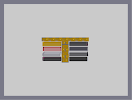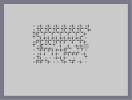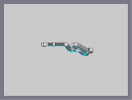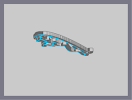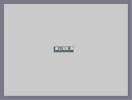Myspace Buttons Another, yet less frustrating, maze. Jag Test Jaguar Maserati Logo C Ya

Pages: (0)

HIM

### I'm thinking

about coming back to this one to finish it.

But not today. Got a 6pg-12pt-dbl.spaced essay on "the effects of Poe's life on his literature" due tomorrow and i have the header of the first page done.XD

But after that, and all of my other class work, i will restart this entire drawing.
Same picture, but different style.

However, the next drawing WILL be my final drawing for N.
I am having too much fun with dan-ball's powder game cus i can draw things in many colors.

Though, to many all believe that i am not using any software to create my work, i WILL record major parts of my work. The hours i spend switching from paint to notepad will be cut out. It will be from start to finish.

Hope you all will like the movie, once i make it and show those people who don't believe in my words that i almost never lie to people online.

Next step...
Getting a GOOD vid. recorder program now. XD

### Come back and finish it!

OMFG IT'S SO BUTIFUL!!!! <CRIES> *cough* *cough* *cough* i see ... a light so pretty oh wait, that's just ur art amazing crazy weird cool icey raw rawsome i could go on forever

### update

* (whispers) I LOVE YOU!!!*

### i know

but i am thinking of doing this over and only doing a 3pt. outline of it

### dude don't even bother

finishing this...
I know the picture, it's big... and by the time you have wasted your life making it, and can submit it you will realise that nmaps doesn't support insanely large amounts of objects anymore... I've tried, and you won't fit this on there.

### omg

How do you make this stuff? It's just amazing! Can't wait to see the finished product. 4.5aved but i'll round up.

### The skull one you are speaking of was made using a program

designed by Eeyore.

And yeah, IMO try something new. These get old fast.

### nvm

i saw your other maps, do you remember that scull i cant remember who made it was created the same way you made the jaguare and the logo, i thing your next step is to make large 3d objects like that one, i think it was 3rd in favorites behind dragon moon

### looks like another logo

guess we wait.Try2make more things other that part screen pic, not that im not trying to say anything bad, your art is beter than most, mix it up and people will come

### dude!

i made a stencil out of that! I was gunna make Nart out of it, but i retired.
I'm not gonna rate this though, even when it's finished... you lack variety, you made the jaguar, that was cool. but you have made pretty much the same style, try something different, not friggin thwumps and bounce blocks.

### Riobe

IDK, what i will do next.

I guess i will try some other tribal thing or try to do a car outline or something.

i relay don't know where i will go next.

### palemoon

Is your avatar one of the dogs from the game cave story?
I love that game.

### i was going to say it if atob did not

would i have the same effect? probably not.

### "but there he goes, abusing his admin privileges again."

You now he's gonna have to comment back on that if he see's this. :P

BTW, do you have any ideas on what your doing next?

### lol

agreed

unfinished = no rate

### I agree with atob

but there he goes, abusing his admin privileges again.

I don't really know what this is gonna be but right now it's just a kinda cool design... like your others but unfinished, nr

### ATOB has a good point

That's why I didn't rate.

### finished product

will be another map. This is just the test that i am doing. The full one will be on its own.

### Very nice so far,

looking forward to the finished result.

### This has 5/5 with 2...

How can you guys rate an unfinished product so highly?

I'm holding off until the finished thing, I'd suggest y'all do the same.

### actually, i dont fave

so i dont know if ill see this or not.. could you hit me up with a link when youre done? id love to see it.

### keep working,

ill rate when its done, i know itll be good.

### This alread looks pretty amazing

But the amazing thing takes a lot of time, that is what makes you art special, because I think you're the only one who can make them. Cant wait to see the finished Dragon.

### I'm amazed

Never new thwump-blue cood be so malleable.

5

### It's cool

It looks like it could turn into a cool cobra. But I'd rather see the dragon. :)